• 关系抽取关系抽取关系抽取 1.PCNNATT-2016 2.ARNOR-2019
$关系抽取$
1.PCNNATT-2016
2.ARNOR-2019


展开全文• 基于深度学习的命名实体识别与关系抽取 摘要：构建知识图谱包含四个主要的步骤：数据获取、知识抽取、知识融合和知识加工。其中最主要的步骤是知识抽取。知识抽取包括三个要素：命名实体识别（NER）、实体关系抽取...
基于深度学习的命名实体识别与关系抽取
作者：王嘉宁 QQ：851019059 Email：lygwjn@126.com 个人网站：http://www.wjn1996.cn
【备注：此博文初次编辑为2018年11月23日，最新编辑为2019年10月24日】夏栀的博客——王嘉宁的个人网站 正式上线，欢迎访问关注：http://www.wjn1996.cn

摘要：构建知识图谱包含四个主要的步骤：数据获取、知识抽取、知识融合和知识加工。其中最主要的步骤是知识抽取。知识抽取包括三个要素：命名实体识别（NER）、实体关系抽取（RE） 和 属性抽取。其中属性抽取可以使用python爬虫爬取百度百科、维基百科等网站，操作较为简单，因此命名实体识别（NER）和实体关系抽取（RE）是知识抽取中非常重要的部分，同时其作为自然语言处理（NLP）中最遇到的问题一直以来是科研的研究方向之一。
本文将以深度学习的角度，对命名实体识别和关系抽取进行分析，在阅读本文之前，读者需要了解深度神经网络的基本原理、知识图谱的基本内容以及关于循环神经网络的模型。可参考本人编写的博文：（1）基于深度学习的知识图谱综述；（2）深度神经网络。
本文的主要结构如下，首先引入知识抽取的相关概念；其次对词向量（word2vec）做分析；然后详细讲解循环神经网络（RNN）、长短期记忆神经网络（LSTM）、门控神经单元模型（GRU）；了解基于文本的卷积神经网络模型（Text-CNN）；讲解隐马尔可夫模型（HMM）与条件随机场等图概率模型（CRF）；详细分析如何使用这些模型实现命名实体识别与关系抽取，详细分析端到端模型（End-to-end/Joint）；介绍注意力机制（Attention）及其NLP的应用；随后介绍知识抽取的应用与挑战，最后给出TensorFlow源码、推荐阅读以及总结。本文基本总结了整个基于深度学习的NER与RC的实现过程以及相关技术，篇幅会很长，请耐心阅读：

一、相关概念
二、序列模型数据
三、循环神经网络
四、循环神经网络的缺陷
五、长短期记忆神经网络（LSTM）与门控神经单元（GRU）
六、长期依赖模型的优化
七、概率图模型（PGM）
八、运用Bi-LSTM和CRF实现命名实体识别
九、卷积神经网络
十、基于文本的卷积神经网络（Text-CNN）的关系抽取
十一、基于依存关系模型的关系抽取
十二、基于远程监督的关系抽取
十三、注意力机制（Attention）
十四、基于注意力机制的命名实体识别与关系抽取
十五、三元组的存储——图形数据库
十六、Tensorflow实现命名实体识别与关系抽取
十七、推荐阅读书籍
十八、项目实例1（面向智慧农业的知识图谱及其应用系统 · 上海 · 华东师范大学数据科学与工程学院）
十九、项目实例2（博主的本科毕业设计&计算教育——智学AI·基于深度学习的学科知识图谱）
二十、总结

一、相关概念
在传统的自然语言处理中，命名实体识别与关系抽取是两个独立的任务，命名实体识别任务是在一个句子中找出具有可描述意义的实体，而关系抽取则是对两个实体的关系进行抽取。命名实体识别是关系抽取的前提，关系抽取是建立在实体识别之后。
1.1 实体与关系
实体是指具有可描述意义的单词或短语，通常可以是人名、地名、组织机构名、产品名称，或者在某个领域内具有一定含义的内容，比如医学领域内疾病、药物、生物体名称，或者法律学涉及到的专有词汇等。实体是构建知识图谱的主要成员。
关系是指不同实体之间的相互的联系。实体与实体之间并不是相互独立的，往往存在一定的关联。例如“马云”和“阿里巴巴”分别属于实体中的人名和机构名，而它们是具有一定关系的。
在命名实体识别和关系抽取之后，需要对所产生的数据进行整合，三元组是能够描述整合后的最好方式。三元组是指（实体1，关系，实体2）组成的元组，在关系抽取任务中，对任意两个实体1和实体2进行关系抽取时，若两者具有关系，则它们可以构建成三元组。例如一句话“马云创办了阿里巴巴”，可以构建的三元组为（“马云”，“创办”，“阿里巴巴”）。
1.2 标注问题
监督学习中有三种问题，分别是分类问题、回归问题和标注问题。分类问题是指通过学习的模型预测新样本在有限类集合中对应的类别；回归问题是指通过学习的模型拟合训练样本，使得新样本可以预测出一个数值；标注问题则是根据输入的序列数据对其用预先设置的标签进行依次标注。
本文的思想便是序列标注，通过输入的序列数据，选择相应的模型对样本进行训练，完成对样本的标注任务。
常用的标注任务包括命名实体识别、词性标注、句法分析、分词、机器翻译等，解决序列标注问题用到的深度学习模型为循环神经网络。
二、序列模型数据
在深度神经网络一文对深度神经网络的分析中已经指出，传统的BP神经网络只能处理长度固定，样本之间相互独立的数据，而对于处理命名实体识别、关系抽取，以及词性标注、情感分类、语音识别、机器翻译等其他自然语言处理的问题中，文本类的数据均以句子为主，而一个句子是由多个单词组成，不同的句子长度不一致，因此对于模型来说，大多数是以单词为输入，而单个词往往没有特定的意义，只有多个词组合在一起才具有一定的含义。例如对于“马云”一词，单个词“马”可能表达的是动物，“云”一词可能表示的是天上飘得云彩，也可以表示“云计算”的云，而“马云”却表示人名。所以这一类数据之间是有关联的。我们对句子级别的数据称为序列模型数据。
对于文本类的序列模型数据，通常是不能直接作为模型的输入数据的，需要进行预处理。
2.1 one-hot向量
将句子中的单词转换为数字的一种方法是采用one-hot向量。例如训练集中有3000个不重复的单词，根据其在词汇表中的排序，可以依次为其编号，例如“a”编号为0，“book”的编号为359，“water”的编号2441。因此这3000个单词都有唯一的编号。
为了能通过向量形式表达，one-hot向量是指除了下标为该单词编号所对应的的值为1以外其他都为0。例如一个集合只有一句话“马云在杭州创办了阿里巴巴”，其中只有11个不重复的词，分别编号0-10，则“马”字的one-hot向量为$[1,0,0,0,0,0,0,0,0,0,0]$，“阿”字的one-hot向量是$[0,0,0,0,0,0,0,0,1,0,0]$。
one-hot向量能够很清楚得为每一个词进行“数值化表示”，将人理解的内容转换为计算机可以理解的内容。
Ps：有关onehot向量的详解：OneHot编码知识点，数据预处理：独热编码（One-Hot Encoding）
2.2 词嵌入向量（word embeddings）
one-hot向量虽然能够简单的表示一个词，但是却存在三个问题：
（1）one-hot向量是稀疏向量，并不能存储相应的信息；
（2）当语料库中包含的词汇很多时（上百万上千万），一个one-hot向量的维度将会很大，容易造成内存不足；
（3）序列模型的数据需要能够体现出词语词之间的关联性，单纯的one-hot向量不能体现出关联性。例如对于词汇“good”和“well”都表示不错的意思，再某些程度上具有相似关联，而one-hot只是简单的编号，并未体现这层相似性。
因此，为了解决one-hot带来的问题，引入词向量概念。
词向量有许多种表达方式，传统的方法是统计该词附近的词出现的次数。基于深度学习的词向量则有word embeddings，其是通过谷歌提出的word2vec方法训练而来。
word2vec方法是将高维度的one-hot向量进行降维，通常维度设置为128或者300，其通过神经网络模型进行训练。基于神经网络的词向量训练有两种模型，分别是CBOW和Skip-Gram模型，如下图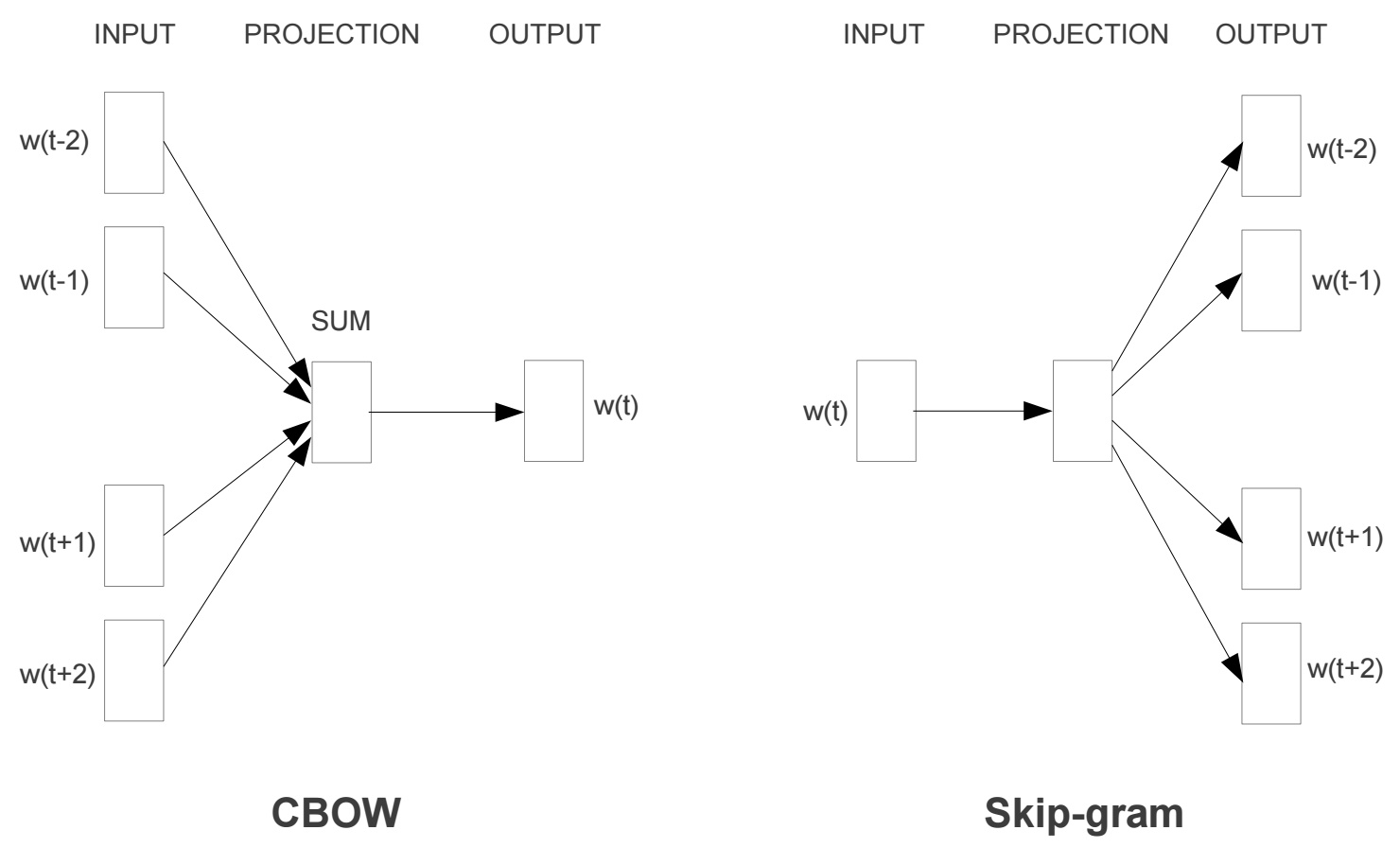（1）CBOW模型是将一个词所在的上下文中的词作为输入，而那个词本身作为输出。通常设置一个窗口，不断地在句子上滑动，每次滑动便以窗口中心的词作为输出，其他词作为输入。基于大量的语句进行模型训练，通过神经网络的梯度下降法进行调参。最终神经网络的权重矩阵即为所有词汇的word embeddings。
（2）Skip-Gram模型与CBOW相反，其随机选择窗口内的一个词的one-hot向量作为输入，来预测其他所有词可能出现的概率，训练后的神经网络的权重矩阵即为所有词汇的word embeddings。
word2vec对训练好的神经网络，需要通过遍历所有词汇表抽取出所有词汇的word embedding，常用的优化模型是哈弗曼树（Hierarchical Softmax）和负采样（Negative Sampling）。
Ps：关于word2vec训练详细解读可参考：如果看了此文还不懂 Word2Vec，那是我太笨，Word Embedding与Word2Vec；关于word2vec的模型优化可参考基于Hierarchical Softmax的模型概述，基于Negative Sampling的模型概述。
三、循环神经网络
循环神经网络是BP神经网络的一种改进，其可以完成对序列数据的训练。根据前面讲解内容，循环神经网络需要能够记住每个词之间的关联性，因为每个词可能会受到之前的词的影响。
3.1 循环神经网络的结构
循环神经网络与BP神经网络不同之处在于其隐含层神经元之间具有相互连接。循环神经网络是基于时间概念的模型，因此对于横向的连接每一个神经元代表一个时间点。模型如图所示：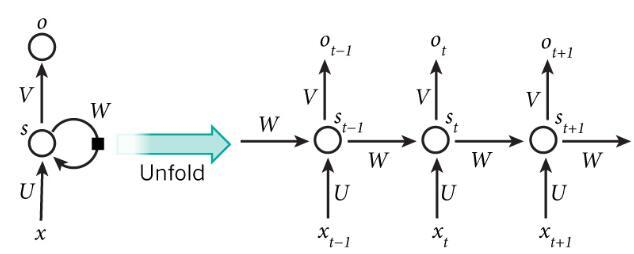对于时刻 $t$ 的输入为 $x_t$，其中 $x_t=\{x_{t1},x_{t2},...\}$ 即为一个word embedding，输入到中心圆圈（隐层状态神经元）的箭头表示一个神经网络，权重矩阵为 $U$，偏向为 $b$，对于时间 $t-1$ 时刻，中心的圆圈已经存在一个值 $s_{t-1}$ ，该圆圈与$t$ 时刻的圆圈的箭头也表示一个神经网络，其权重矩阵为 $W$，偏向也为 $b$ 。循环神经网络的关键即为某一时刻 $t$ 的隐层状态神经元的值不仅取决于当前的输入，也取决于前一时刻的隐层状态，即为：
$s_{t}=f(Ws_{t-1}+Ux_t+b)$
其中$f(·)$表示激活函数。
对于网络的输出部分，循环神经网络输出个数与输入数量一致，时刻 $t$ 的输出为：
$\hat y_t = o_t=softmax(Vs_t+c)$
由于对于序列模型来说，数组的长度是不一致的，即循环神经网络的输入神经元长度是不确定的，因此各个神经网络采用了共享参数 $W，U，V，b和c$。
循环神经网络的剖面图如下图，该图能够比较直观的认识循环神经网络的空间结构（图片为转载，可忽略里面的参数）：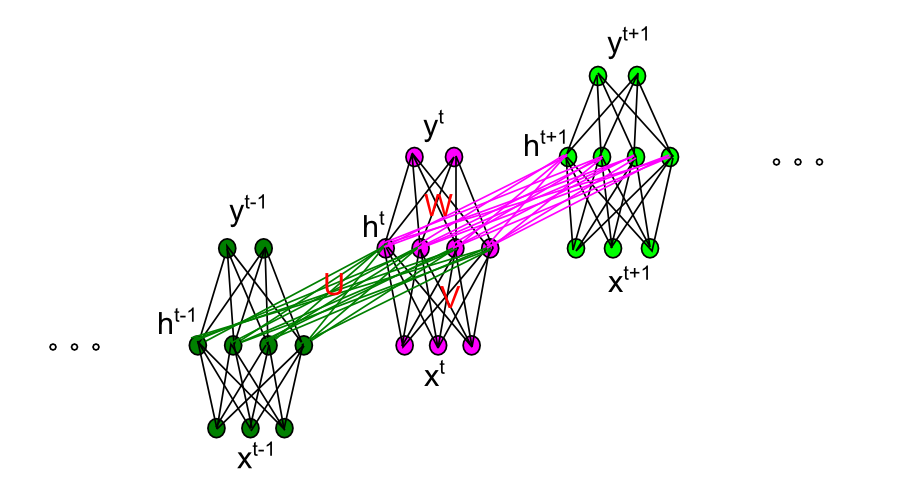3.2 循环神经网络的训练
循环神经网络的训练与传统的BP神经网络训练方法一样，分为前向传播和反向传播，前向传播的公式为：
$s_{t}=f(Ws_{t-1}+Ux_t+b)$
$\hat y_t = o_t=softmax(Vs_t+c)$
假设损失函数为 $L$。反向传播采用BPTT（基于时间的反向梯度下降）算法计算各个参数的梯度。输出层的神经网络梯度下降与BP神经网络的一样。而对于隐含层状态神经元部分，由于其在前向传播过程中的值来自于两个方向，同时又流向另外两个方向，因此在反向传播过程中，梯度将来自当前时刻的输出和下一时刻的状态，同时梯度流向该时刻的输入和上一时刻的状态，如图（$E_t=o_t$）：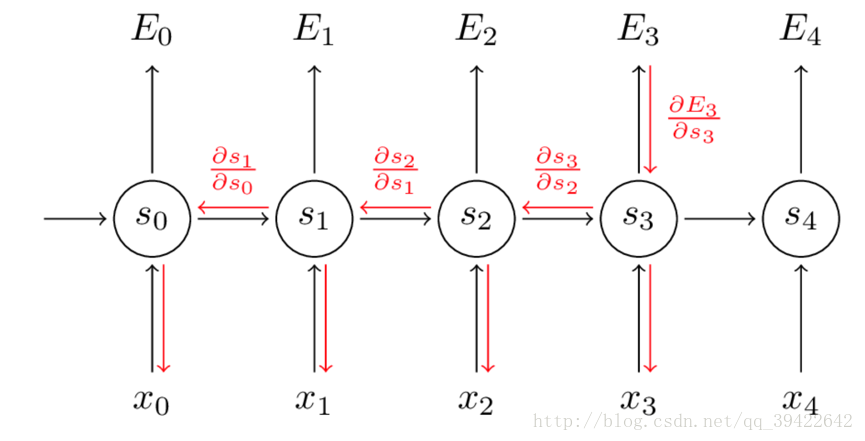因此，假设时间长度为 $n$ ，对于某一时刻 $t(0，隐层状态神经元的梯度将是不断累加的。下面给出循环神经网络的参数 $W，U，V，b，c$ 的梯度：
$\frac{\partial L}{\partial c}=\sum_t (\frac{\partial o_t}{\partial c})^T\frac{\partial L}{\partial o_t}=\sum_t \frac{\partial L}{\partial o_t}$
$\frac{\partial L}{\partial b}=\sum_t (\frac{\partial s_t}{\partial b_t})^T\frac{\partial L}{\partial s_t}=\sum_t diag(1-(s_t)^2)\frac{\partial L}{\partial s_t}$
$\frac{\partial L}{\partial V}=\sum_t \sum_i (\frac{\partial L}{\partial o_{ti}})^T\frac{\partial o_{ti}}{\partial V}=\sum_t \frac{\partial L}{\partial o_t}s_t^T$
$\frac{\partial L}{\partial W}=\sum_t (\frac{\partial L}{\partial s_{ti}})^T\frac{\partial s_{ti}}{\partial W_t}=\sum_t diag(1-(s_t)^2)\frac{\partial L}{\partial s_t}s_{t-1}^T$
$\frac{\partial L}{\partial U}=\sum_t (\frac{\partial L}{\partial s_{ti}})^T\frac{\partial s_{ti}}{\partial U_t}=\sum_t diag(1-(s_t)^2)\frac{\partial L}{\partial s_t}x_{t}^T$
由于循环神经网络的共享参数机制，使得网络可以用很少的参数来构建一个大的模型，同时减少了计算梯度的复杂度。
Ps：关于BPTT算法详细推导，可参考博文：基于时间的反向传播算法BPTT（Backpropagation through time），书籍：人民邮电出版社的《深度学习》233页。
3.3 其他类型的循环神经网络
上述的循环神经网络结构是标准的模型，对于不同的应用会产生不同的模型。在基于循环神经网络的情感分类问题中，模型的输入是长度不一的句子，而模型的输出往往只有一个（是积极的还是消极的评论），因此这属于多对一模型，如图：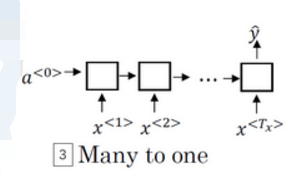对于音乐的生成问题，输入的是一段声音，希望模型输出对于的音符，这种模型为一对多，如图：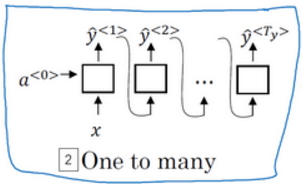对于基于循环神经网络的机器翻译问题，输入的是不定长的待翻译句子，而输出的也是不定长的新句子，即模型的输入与输出长度不一致，这一类属于多对多模型，通常也叫做编码解码器，如图：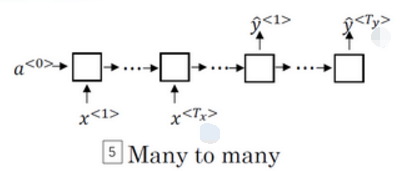3.4 双向循环神经网络
在命名实体识别问题中，输入的数据是一个句子，往往一个句子中的词不仅受到前面词的影响，同时也可能受到后面的词的影响，例如句子“王小明是班级上学习最好的同学”中之所以可以识别出人名实体“王小明”，是因为其后的关键词“同学”，而该实体前面没有词可以提供识别的依据。普通的循环神经网络仅考虑了前一时刻的影响，却未考虑后一时刻的影响，因此需要引入了双向循环神经网络模型。
双向循环神经网络的结构如下：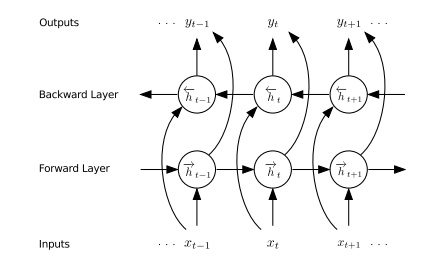其由输入层、前向隐含层、后向隐含层和输出层组成。输入层输入序列数据，前向隐含层的状态流向是顺着时间，后向隐含层的状态流向是逆着时间的，因此前向隐含层可以记住当前时刻之前的信息，而后向隐含层可以记住当前时刻未来的信息。通过输出层将前向、后向隐含层的向量进行拼接或者求和。
双向循环神经网络的前向传播式子为：
$\overrightarrow{h_t}=f_F(U_Fx_t+W_F\overrightarrow{h_{t-1}}+b_F)$
$\overleftarrow{h_t}=f_B(U_Bx_t+W_B\overleftarrow{h_{t-1}}+b_B)$
$h_t=[\overleftarrow{h_t},\overrightarrow{h_t}] 或 h_t=\frac{1}{2}(\overleftarrow{h_t}+\overrightarrow{h_t})$
$\hat y_t=o_t=softmax(Vh_{t}+c)$
双向循环神经网络的梯度下降法仍然使用BPTT算法，对于前向和后向隐含层，梯度的流向是互不干扰的，因此可以用普通的循环神经网络梯度下降的方法对前向和后向分别计算。
3.5 深度循环神经网络
循环神经网络的结构还是属于浅层模型，可以观察其结构图，只有两层网络组成。为了提高模型的深度，一种深度循环神经网络被提出，其结构如图所示：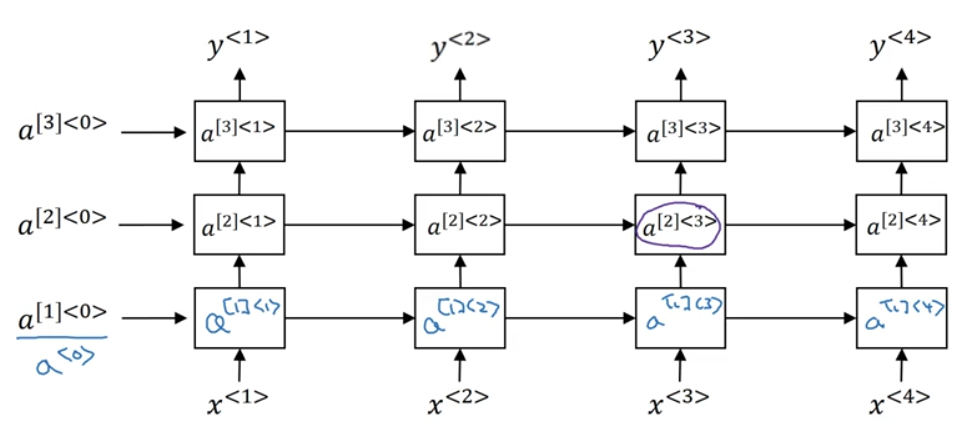可知每一层便是一个循环神经网络，而下一层的循环神经网络的输出作为上一层的输入，依次进行迭代而成。
深度循环神经网络的应用范围较少，因为对于单层的模型已经可以实现对序列模型的编码，而深层次模型会造成一定的过拟合和梯度问题，因此很少被应用。
四、循环神经网络的缺陷
在命名实体识别任务中，通过训练循环神经网络模型，实体识别的精度往往可以达到60%-70%，同时以其共享参数的机制，构建一个较大的循环神经网络不需要像BP网络那样需要庞大的参数，因此模型的训练效率也大大提高。
但是循环神经网络有两个致命的缺陷，在实验中经常遇到：
（1）容易造成梯度爆炸或梯度消失问题。当序列长度为100时，第1个输入的梯度在传递100层之后，可能会导致指数上升或者指数下降。
（2）对于较长的序列，模型无法能够长期记住当前的状态。例如对于句子“这本书，名字叫平凡的世界，白色封皮，有一个书签夹在里面，旁边放着一支笔，…，是我的”，若要提取出有效的信息“这本书是我的”，需要长久的记住“这本书”，直到遇到“是我的”为止，而对于省略号完全可以无限的长。因此普通的循环神经网络无法记住这么久。
因此为了解决这两个问题，需要对循环神经网络做出改进，使得其能够长期记住某一状态。
五、长短期记忆神经网络（LSTM）与门控神经单元（GRU）
为了解决循环神经网络的缺陷，引入门控概念。通过设置门结构来选择性的决定是否记忆或遗忘。
5.1 长短期记忆神经网络（LSTM）
长短期记忆神经网络（LSTM）巧妙的运用的门控概念，实现了可以长期记忆一个状态。LSTM的模型结构与循环神经网络结构是一样的，如图：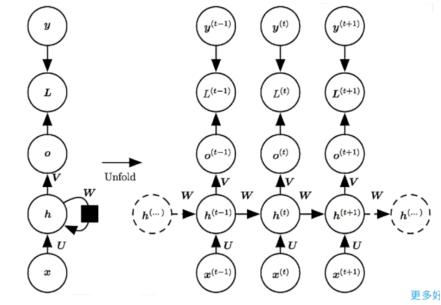不同的是隐含层的部分不是简单的求和。隐含层的部分又称为LSTM单元，如图：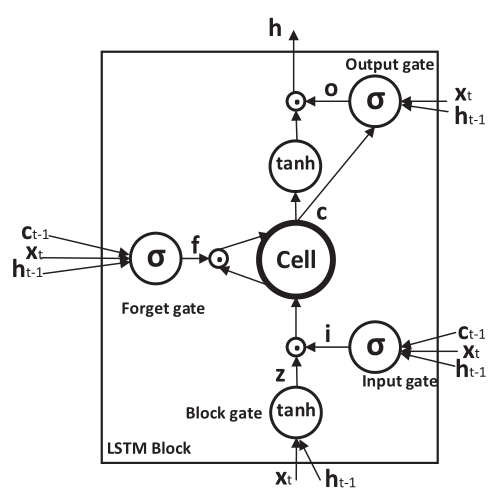其主要由三个门控组成，分别是遗忘门、输入门和输出门，中间的Cell称为记忆细胞，用来存储当前的记忆状态。
（1）遗忘门：遗忘门的作用是决定记忆细胞中丢弃什么信息。采用的是sigmod激活函数，其数据来源于当前的输入、上一时刻的隐层状态以及上一时刻的记忆细胞，前向传播的公式为：
$f_t=\sigma(W_{xf}x_t+W_{hf}h_{t-1}+W_{cf}c_{t-1}+b_f)$
$f_t$ 值取值为0或1，0表示完全丢弃，1表示完全保留。
（2）输入门：输入门决定了需要新增的内容。采用的是sigmod激活函数，前向传播公式为：
$i_t=\sigma(W_{xi}x_t+W_{hi}h_{t-1}+W_{ci}c_{t-1}+b_i)$
$i_t$ 的值为0或1，0表示不添加当前的内容，1表示新增当前的内容，而待新增的内容取决于当前时刻的输入以及上一时刻的隐含状态（这与普通的循环神经网络是一致的），待新增内容记为 $z_t$：
$z_t=tanh(W_{xc}x_t+W_{hc}h_{t-1}+b_c)$
Ps：遗忘门与输入门的计算公式中输入的数据都是一模一样的，区别两者功能的便是相应的权重矩阵和偏向。
（3）记忆细胞：记忆细胞内存储着已经记住的内容，当确定当前时刻是否保留过去的记忆（即 $f_t$ 的取值）和是否记住新的内容（即 $i_t$ 的取值），于是更新记忆细胞，公式为：
$c_t=f_tc_{t-1}+i_tz_t$
更新细胞的公式可以这么理解：$c_{t-1}$ 表示 $t-1$ 时刻LSTM模型记住的内容，当在 $t$ 时刻时将面临两个问题，是否继续记住之前（$t-1$ 时刻）的内容？是否需要记住当前新的内容？因此将有四种情况：
（1）当 $f_t=0$ 且 $i_t=0$ 时，$c_t=0$，即忘记过去全部内容且不记住新的内容；
（2）当 $f_t=0$ 且 $i_t=1$ 时，$c_t=z_t$ ，即忘记过去全部内容，但记住新的内容；
（3）当 $f_t=1$ 且 $i_t=0$ 时，$c_t=c_{t-1}$，即保留之前的内容，对新的内容不予理睬；
（4）当 $f_t=1$ 且 $i_t=1$ 时，$c_t=c_{t-1}+z_t$，即既保留之前的内容，又记住新的内容。
Ps：而在实际的实验中，因为sigmod函数并不是二值的（即其是在0-1之间的一个值），因此对于 $f_t$ 和 $i_t$ 实际上是分别决定了保留记忆过去内容和选择记住新的内容的多少，例如 $f_t=1$ 则表示保留全部过去内容，$f_t=0.5$ 则表示忘记过去的一半内容，或表示为淡化过去的记忆。
（4）输出门：输出门则是决定输出什么内容，即对于当前时刻 $t$ ，若 $o_t=0$ 则表示不输出，若 $o_t=1$ 则表示输出：
$o_t=tanh(W_{xo}x_t+W_{ho}h_{t-1}+W_{co}c_t+b_o)$
待输出的内容则是：
$h_t=o_ttanh(c_t)$
其中 $tanh(c_t)$ 是对当前时刻记忆细胞内记住的内容进行处理使其值范围在-1至1之间。
因此总结LSTM模型的原理：在第 $t$ 时刻时，首先判断是否保留过去的记忆内容，其次判断是否需要新增内容，更新记忆细胞之后再判断是否需要将当前时刻的内容输出。
LSTM的梯度下降法仍然使用BPTT算法（因为每个门其实就是一个神经网络），梯度下降法与循环神经网络的思路和原理是一样的，此处不再推导。
5.2 双向长短期记忆神经网络（Bi-LSTM）
与循环神经网络一样，单向的模型不能够记住未来时刻的内容，因此采用双向模型，双向模型如图所示：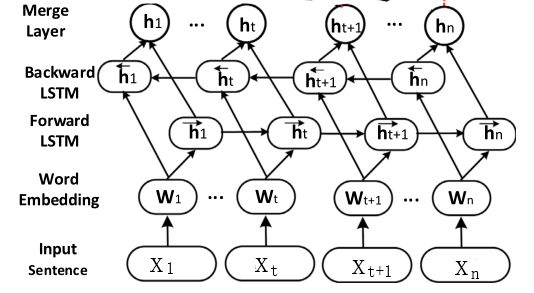Bi-LSTM的结构与Bi-RNN模型结构一样。同一时刻在隐含层设置两个记忆单元（LSTM unit），分别按照顺时间（前向）和逆时间（后向）顺序进行记忆，最后将该时刻两个方向的输出进行拼接，即：
$h_t=[\overleftarrow{h_t},\overrightarrow{h_t}]$
在诸多学术论文中，对命名实体识别最常用的便是Bi-LSTM模型，该模型实体识别的精度通常高达80%。
5.3 门控神经单元（GRU）
门控神经单元（GRU）是LSTM的一个变种，其简化了LSTM结构，其只有重置门和更新门两个门结构，GRU单元结构如图所示：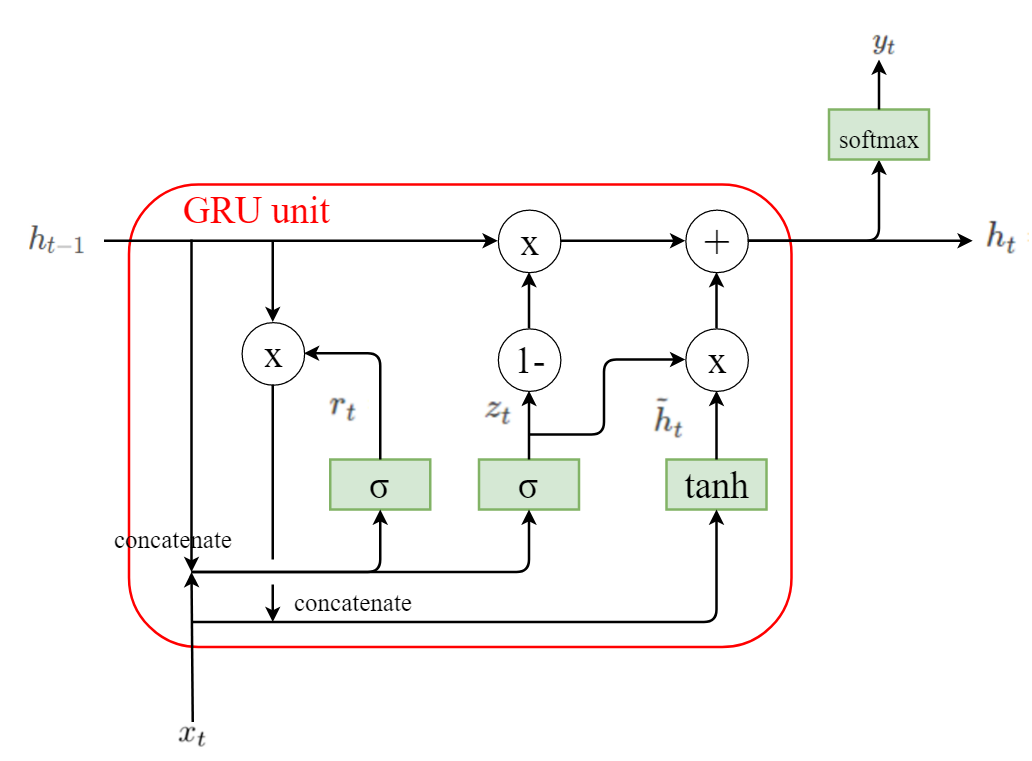（1）重置门：决定是否重置当前的记忆， $r_t$ 的取值为0或1，0表示重置，1表示不重置：
$r_t=σ(Wr[h_{t−1},x_t]+b_r)$
（2）更新门：决定是否更新当前的心内容，待更新的内容为：
$\hat h_t=tanh⁡(W_h[r_th_{t−1},x_t]+b_h)$
更新门为 $z_t$ ，取值为0或1：
$z_t=σ(Wz[h_{t−1},x_t]+b_z)$
于是有：
$h_t=(1−z_t)h_{t−1}+z_t\hat h_t$
可知当 $z_t=0$ ， $h_t=h_{t-1}$ ，即保留过去的内容，即不更新；当 $z_t=1$ ， $h_t=\hat h_t$ 即更新当前时刻的内容。
（3）模型输出：
$y_t=σ(W_oh_t+b_y)$
GRU相比LSTM只有两个门结构，运算方面更加快，同时也能实现长期记忆。例如对于句子“The cat,which already ate …,was full.”，需要模型能够正确表示为“was”而不是“were”，当处于单词“cat”时刻时，设置重置门为1，更新门为1，此时模型 $h_t$ 为“cat”（单数），然后经过“which”、“already”…，均设置重置门和更新门为0，此时 $h_t$ 的值始终是 “cat”（单数），直到遇到“was”为止。
GRU的训练仍然为BPTT算法实现梯度下降法调参。
Ps：GRU详解参考：GRU神经网络，门控循环单元（GRU）的基本概念与原理
六、长期依赖模型的优化
在模型的训练过程中，容易出现梯度爆炸或梯度衰减的问题，而对于LSTM这种高维度非线性模型，容易造成不同大小梯度之间的骤降，即参数在很小的变化范围内代价函数的梯度呈现指数级别的“爆炸”。假设对于代价函数 $J(W,b)$ 的梯度图如图所示：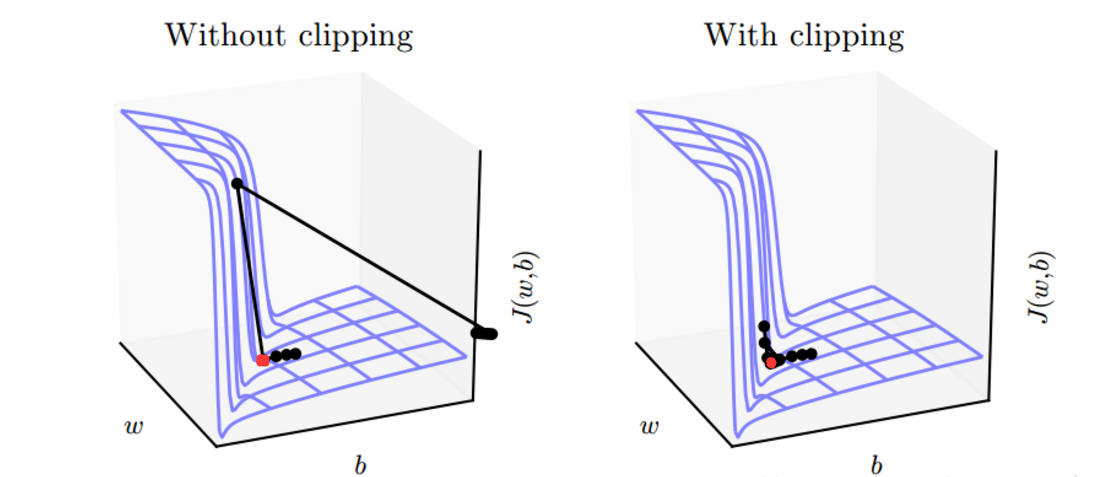对于含有陡峭悬崖的梯度模型，需要进行梯度截断（Gradient Clipping）。其中红色的点为最优值的位置，如果使用梯度截断法（右图），则可以使梯度在接近悬崖时降低步伐（学习率衰减），如果不使用梯度截断（左图），则可能由于过大的学习率使当前的参数被“抛出”曲面。
截断梯度法有两种策略，一种是在参数更新前，逐元素地截断小批量产生的参数梯度；另一种策略是在参数更新前截断梯度 $g$ 的范数 $||g||$（$g$ 表示待更新的参数，例如权重矩阵 $W$ 和偏向 $b$）。设 $v$ 是范数的上界，则梯度截断的参数更新表示为
$g:=\frac{gv}{||g||}$
其中 $||g||>v$ 。
梯度截断法很好的解决了梯度爆炸问题，但对于梯度衰减问题则无济于事。对于LSTM模型，梯度衰减容易导致记忆内容的丢失，因此为了能够捕获长期依赖，需要优化模型的结构：
（1）修改门结构；
（2）修改损失函数的正则项：引导信息流的正则化
七、概率图模型（PGM）
在基于深度学习的命名实体识别中，使用RNN、LSTM或GRU模型是对序列数据的一种编码（encoding），虽然RNN、LSTM和GRU的输出数据也表示对实体标注的预测，但往往会出现错误。例如通常在命名实体识别中使用“BIES”表示实体词中每个单词的相对位置，其中“B”表示位于实体词的第一个位置，“I”表示位于实体词的中间位置，“E”表示位于实体词的最后一个位置，“S”表示该实体词只有一个单词（例如实体“华东师范大学”的标注序列为“BIIIIE”）。而对于RNN、LSTM的输出只会单纯的输出其是否是实体，而并未考虑相对位置，即模型可能对“华东师范大学”实体输出“BBBBBB”，显然这是个错误的。因此需要引入解码器（decoding）。
常用的解码器可以是LSTM进行解码，在部分论文中也使用LSTM进行解码，但常用的解码器是基于概率图模型的隐马尔可夫模型和条件随机场模型。
7.1 概率图模型概念
概率图模型是通过图结构直观的表现出各个随机变量之间的依赖关系。图结构如图所示：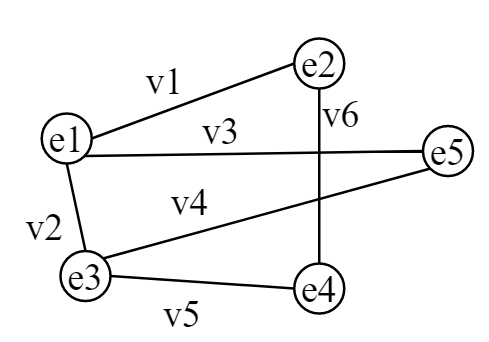图 $G(V,E)$ 中有 $n$ 个结点 $V=\{v_1,v_2,...,v_n\}$ 分别表示各个随机变量， $X=\{X_{v_1},X_{v_2},...X_{v_n}\}$ ；边 $E=\{e_1,e_2,...,e_m\}$ 则表示其相连的两个结点表示的随机变量的依赖关系。概率图模型分为两种：有向概率图模型和无向概率图模型。有向概率图包括贝叶斯网络，隐马尔可夫模型，无向图包括条件随机场等。
7.2 贝叶斯网络
贝叶斯网络又称信念网络或因果网络，其属于有向无环图，例如对于结点 $v1$ 和 $v2$ 分别表示随机变量 $X_1$ 和 $X_2$ ，则随机变量 $X_2$ 的概率为 $p(X_2|X_1)$ ，即变量 $X_1$ 是 $X_2$ 的因。对于没有边相连的结点表示的随机变量，则两者是相互独立的。
&emsp在概率论中，贝叶斯定理表示的条件概率与各个变量之间的关系，即：
$p(A|B)=\frac{p(A)p(B|A)}{p(B)}$
假设图 $G(V,E)$ 中有5个结点 $V=\{v_1,v_2,v_3，v_4，v_5\}$ 分别表示随机变量  $X=\{X_{v_1},X_{v_2},X_{v_3},X_{v_4},X_{v_5}\}$ ，有向边如图所示：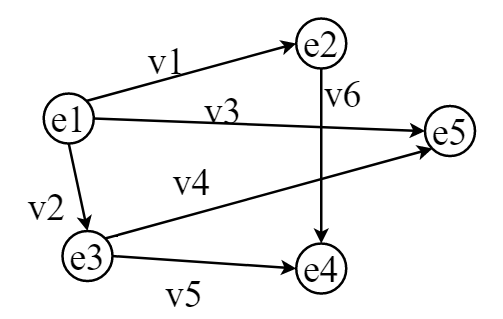因此可知变量 $X_1$ 是所有变量的因，各个变量的条件概率为：
$p(X_1)=p_1$
$p(X_1,X_2)=p(X_2|X_1)p(X_1)=p_{21}·p_1$
$p(X_1,X_3)=p(X_3|X_1)p(X_1)=p_{31}·p_1$
$p(X_1,X_2,X_3,X_4)=p(X_1,X_3,X_4)p(X_1,X_2,X_4)$
$=p(X_4|X_1,X_3)p(X_1,X_3)·p(X_4|X_1,X_2)p(X_1,X_2)=p_{413}p_{31}·p_{412}p_{21}·p{_1}{^2}$
$p(X_1,X_3,X_5)=p(x_5|x_1,x_3)p(x_1,x_3)=p_{513}p_{31}p_1$
因此有：
$p(X_1,X_2,X_3,X_4,X_5)=p(X_1,X_2,X_3,X_4)·p(X_1,X_3,X_5)=p_{513}p_{413}p_{412}p_{21}p{_{31}}{^2}p{_{1}}{^2}$
7.2 隐马尔可夫模型（HMM）
隐马尔可夫模型是一种特殊的贝叶斯网络，各个随机变量之间的依赖关系并不像图结构那样错综复杂，而是单纯的一条链式结构，因此称为隐马尔可夫链，如图所示：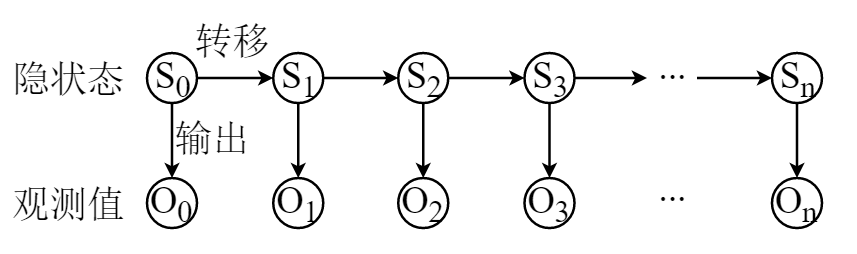$S=\{S_0,S_1,...,S_n\}$ 表示隐含状态序列，其中 $S_t$ 表示第 $t$ 时刻的某一状态。隐马尔可夫模型的假设指出，$t$ 时刻状态仅受 $t-1$ 时刻影响，与其他无关（即 $p(S_t|S_0,S_1,..,S_{t-1})=p(S_t|S_{t-1})$），因此 $t-1$ 时刻与 $t$ 时刻之间存在一个有向边，表示状态的转移，用矩阵 $A$ 表示。每一个时刻的状态都将对应一个输出，且该时刻的输出仅与当前时刻的隐含状态有关，输出的值即为观测值，输出矩阵为 $B$。
隐马尔可夫模型所表达的含义即多个随机变量含有隐含状态（内因）以及它们对应的外在表现（观测序列）。隐马尔可夫模型解决的问题主要包括评估问题、解码问题和学习问题。
在命名实体识别中，主要应用的是解码问题。关于解码问题主要指根据已知的观测序列，推测最有可能的隐状态序列。即已知 $O=\{O_1,O_2,...,O_n\}$来推测 $S$，通过初始化隐状态的转移概率矩阵以及各个观测值为某个状态的概率，可构建起若干条状态路径，每一条路径对应一个评分值，因此通过选择最大评分值对应的路径即为预测的隐含状态序列。在解决若干条路径的最值问题，可以参考最短路径算法或者动态规划算法来解决。
Ps：隐马尔可夫模型的原理以及通过例子来推导解码过程可参考：HMM超详细讲解+代码，隐马尔可夫模型。
7.3 条件随机场模型（CRF）
条件随机场(CRF)是给定一组输入序列条件下另一组输出序列的条件概率分布模型，在自然语言处理中得到了广泛应用。对于命名实体识别这一类的序列标注问题，通常采用的是线性条件随机场（linear-CRF），模型如图所示：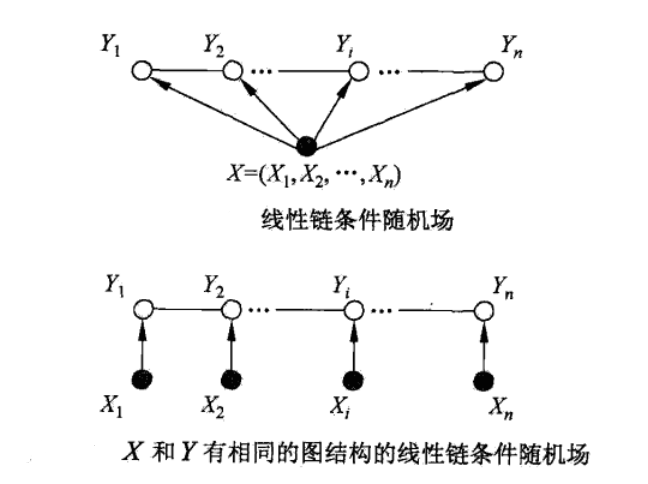线性条件随机场仍满足隐马尔可夫模型（HMM），在HMM的基础上引入特征函数 。特征函数分别为$s_l$ 与 $t_k$。
（1）$s_l(y_i,x,i) (l=1,2,...L)$表示当前的状态特征，其只与当前状态有关，其中 $L$ 是定义在该节点的节点特征函数的总个数， $x$ 表示观测序列， $i$ 是当前节点在序列的位置。
（2）$t_k(y_{i-1},y_i,x,i)$ 表示当前时刻的转移特征，即由 $k-1$ 时刻转移至 $k$ 时刻的特征函数，因此当前的状态特征与之前时刻的状态有关。
linear-CRF的参数化形式如下：
$P(y|x) = \frac{1}{Z(x)}exp\Big(\sum\limits_{i,k}\lambda_kt_k(y_{i-1},y_i, x,i) +\sum\limits_{i,l}\mu_ls_l(y_i, x,i)\Big)$
其中 $\lambda_k$ 和 $\mu_l$ 为权重系数， $Z(x)$ 为归一化因子。
线性条件随机场还有其他表示形式，包括参数化形式（即上面的表达式），也有矩阵形式，具体可参考李航的《统计学习方法》194-199页。
条件随机场解决的问题也为三个：评估问题、解码问题和学习问题。在命名实体识别、词性标注等序列标注问题上，普遍运用CRF实现解码，并通过Viterbi算法求得最大概率的序列。
7.4 概率图模型解决命名实体识别
通过运用HMM和CRF模型实现命名实体识别，需要首先初始化相应的参数，例如对于HMM模型，需要初始化转移状态矩阵 $A$、观测序列与状态的关联（混淆矩阵） $B$ ，以及初始化状态概率（即第一个时刻为某一状态的概率）；对于CRF模型，需要初始化两个特征函数以及其对应的系数。其次通过观测序列和状态序列对这些参数进行训练，训练即属于三个问题中的学习问题。最后通过已学习的模型，通过训练集样本进行解码测试。
现如今非常常用的模型是Bi-LSTM+CRF，即应用Bi-LSTM实现对序列（一个句子）进行编码（encoding），使得该编码保存了整个语句的前后关系，其次将Bi-LSTM的输出通过CRF进行解码，已获取最为可能的序列标注。
八、运用Bi-LSTM和CRF实现命名实体识别
前面讲解了双向长短期记忆神经网络以及条件随机场概率图模型，本节将运用Bi-LSTM与CRF来实现命名实体抽取。
8.1 数据获取与处理
数据集可以采用自定义标注的数据，但这一类数据往往会存在很多缺陷，因此，在实验中通常使用公开训练集。
常用的公开训练集有ACE04，ACE05，可以用来完成词性标注（命名实体识别便属于一种词性标注问题）。训练集中包含成千上万个完整的句子，主要以英文句子为主。对于词性标注问题，还将对于一个标注序列。数据通常是以JSON格式保存，在读取数据时需要进行JSON解析。
获取数据后，该数据不能直接作为计算机的输入，需要转化为词向量。词向量可以用自己的语料库使用神经网络（CBOW或Skip-Gram模型）进行训练。实验常用谷歌训练好的词向量，其包含了上千万个语料库，相比自己训练的更加完善。
下面以一句话“马云在杭州创办了阿里巴巴”为例，分析Bi-LSTM+CRF实现命名实体识别的训练与预测过程。实体给定范围的JSON表示为：
{
‘o’:0,
‘B-PER’:1,
‘I-PER’:2,
‘B-LOC’:3,
‘I-LOC’:4,
‘B-ORG’:5,
‘I-ORG’:6
}
该句子每个字对于的语料库（假设共3000字）中的编号假设为：
{
‘马’ : 1683,
‘云’ : 2633,
‘在’ : 2706,
‘杭’ : 941,
‘州’ : 2830,
‘创’ : 550,
‘办’ : 236,
‘了’ : 1436,
‘阿’ : 1,
‘里’ : 1557,
‘巴’ : 213,
}
因此该句子的one-hot向量应该为：{1683,2633,2706,941,2830,550,236,1436,1,1557,213}。其次将该one-hot向量与词嵌入矩阵word embeddings相乘，得到该句子每个字对于的词向量，因此该句子将得到一个句子向量，用 $x$ 表示，假设word embedding对每个词的维度为300（通常实验都设定为300），则 $x$ 的长度也为300。
Ps：通常在训练数据集时，假设一个数据集中有1000个句子，通常采用的是mini-batch法进行训练，即将1000个句子分为若干组（假设分为10组），则每组将平均随机分到batch_size个句子（即每组100个句子），其次将这一组内的句子进行合并。因为每个句子长度不一致，所以取最长的句子为矩阵的列数，其他句子多余的部分则填充0。
8.2 LSTM单元编码
获取该句子的向量后，便将其放入LSTM的的输入层（论文中也多称为input layer或者embedding layer），每个输入神经元对应一个字的词向量，正向传播则从第一个字“马”开始，随着时间推移一直到“巴”。
每个时刻 $t$ 对于的字 $x_t$ 通过前向传播和后向传播并拼接得到 $h_t$，其次得到 $\hat y_t$，该值即为当前时刻 $t$ 对应的7个标签中每个标签预测的概率。例如对于“马”字，$\hat y_t=[0.031,0.305,0.219,0.015,0.129,0.133,0.168]$ ，最大的值为0.305，对应于下标1，即标签“B-PER”。
8.3 CRF解码
在CRF中要解决的问题之一是解码问题，对于 $\hat y_t$ 的结果不一定完全符合输出规则，因此需要将其按照输出规则进行解码。输出规则则体现在CRF中的超参数和参数。例如对于 $t=5$ 时刻，字为“州”，对应的 $\hat y_t=[0.085,0.113,0.153,0.220,0.207,0.108,0.114]$，可知最大的值对应下标表示的标签为“B-LOC”，虽然成功的预测了其属于地区这一类实体，但很显然应该是“I-LOC”。因此将该输出概率向量做下列计算：
$P(y_t|\hat y_t) = \frac{1}{Z(x)}exp\Big(\lambda_kt_k(\hat y_{t-1},y_t, x,t) +\mu_ls_l(y_t, x,t)\Big)$
然后对其他词按照该式子进行计算，通过维特比算法求出最大值，即对应的序列中，“州”字的概率向量可能变为：$\hat y_t=[0.085,0.113,0.153,0.207,0.220,0.108,0.114]$ 。
应用Bi-LSTM和CRF模型的命名实体识别在论文《Bidirectional LSTM-CRF Models for Sequence Tagging》中被提出，可参考该论文，点击一键下载。
九、卷积神经网络
卷积神经网络是神经网络的另一个演化体，其通常用于图像处理、视频处理中，在自然语言处理范围内，常被用来进行文本挖掘、情感分类中。因此基于文本类的卷积神经网络也广泛的应用在关系抽取任务中。
9.1 卷积运算
卷积神经网络主要通过卷积运算来实现对多维数据的处理，例如对一副图像数据，其像素为6x6，通过设计一个卷积核（或称过滤器）filter来对该图形数据进行扫描，卷积核可以实现对数据的过滤，例如下面例子中的卷积核可以过滤出图像中的垂直边缘，也称为垂直边缘检测器。
例如假设矩阵：
$\begin{bmatrix} 3 & 0 & 1 & 2 & 7 & 4 \\ 1 & 5 & 8 & 9 & 3 & 1 \\ 2 & 7 & 2 & 5 & 1 & 3 \\ 0 & 1 & 3 & 1 & 7 & 8 \\ 4 & 2 & 1 & 6 & 2 & 8 \\ 2 & 4 & 5 & 2 & 3 & 9 \\ \end{bmatrix}$
表示一个原始图像类数据，选择卷积核（垂直边缘检测器）：
$\begin{bmatrix} 1 & 0 & -1 \\ 1 & 0 & -1 \\ 1 & 0 & -1 \\ \end{bmatrix}$
然后从原始图像左上方开始，一次向右、向下进行扫描，扫描的窗口为该卷积核，每次扫描时，被扫描的9个数字分别与卷积核对应的数字做乘积（element-wise），并求这9个数字的和。例如扫描的第一个窗口应该为：
$3*1+1*1+2*1+0*0+5*0+7*0+1*(-1)+8*(-1)+2*(-1)=-5$
则最后生成新的矩阵：
$\begin{bmatrix} -5 & -4 & 0 & 8 \\ 10 & -2 & 2 & 3 \\ 0 & -2 & -4 & -7 \\ -3 & -2 & -3 & -16 \\ \end{bmatrix}$
以上的事例称为矩阵的卷积运算。在卷积神经网络中，卷积运算包括如下几个参数：
（1）数据维度：即对当前需要做卷积运算的矩阵的维度，通常为三维矩阵，维度为 $m*n*d$ 。
（2）卷积核维度：即卷积核的维度，记为 $f*f*d$ ，当d为1时，为二维卷积核，通常对二维矩阵进行卷积运算，当 $d>1$ 时为三维卷积核，对图像类型数据进行卷积运算。
（3）卷积步长stride：即卷积核所在窗口在输入数据上每次滑动的步数。上面的事例中明显步长为1。
（4）数据填充padding：在卷积操作中可以发现新生产的矩阵维度比原始矩阵维度变小，在一些卷积神经网络运算中，为了保证数据维度不变，设置padding=1，对原始矩阵扩充0。这种方式可以使得边缘和角落的元素可以被多次卷积运算，也可以保证新生成的矩阵维度不变。可以计算出每个维度应该向外扩充 $f-1$ 或 $d-1$。
9.2 卷积神经网络的结构
卷积神经网络的基本结构如图所示：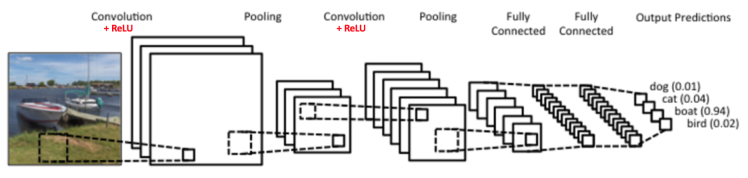其主要有输入层、卷积层、池化层、全相连接层和输出层组成，其中卷积层与池化层通常组合在一起，并在一个模型中循环多次出现。
（1）输入层：输入层主要是将数据输入到模型中，数据通常可以是图像数据（像素宽*像素高*三原色（3）），也可以是经过处理后的多维数组矩阵。
（2）卷积层：卷积层主要是对当前的输入数据（矩阵）进行卷积运算，9.1节简单介绍了卷积运算，下面对卷积运算进行符号化表示：
设当前的输入数据为图像 $G(G\in\mathbb R^{m*n*d})$ ，其中 $m$ 表示 $G$ 的宽， $n$ 表示 $G$ 的高， $d$ 表示 $G$ 的层数。
设卷积神经网络有2次卷积和池化操作（如上图），两次卷积操作分别有 $c^{(s)}(s=1,2)$ 个卷积核 $C^{(s,t)}(t\in\mathbb [1,c^{(s)}])$（每个卷积核各不相同，不同的卷积核可以对原始图像数据进行不同方面的特征提取），每个卷积核的维度设为 $f_s*f_s*d$ （卷积核维度一般取奇数个，使得卷积核可以以正中间的元素位置中心对称，卷积核的层数需要与上一轮输出的数据层数一致），因此对于第 $s$次中的第 $t$ 个卷积核的卷积操作即为：
$G_{pq}^{(s,t)}=\sum_{k=1}^{d}\sum_{i=p}^{p+f_s}\sum_{j=q}^{q+f_s}C^{(s,t)}_{ijk}*P_{pq}^{(s-1,t)}$
其中 $pq$ 表示卷积后的矩阵的第p行第q列， $P_{pq}^{(s-1,t)}$ 表示第 $s-1$ 次卷积池化操作后的池化层值，$P^{(0,1)}$ 即为原始图像数据 $G$。
卷积运算后，将生成 $c^{(s)}$ 个维度为 $\lfloor \frac{m+2*padding-f_s}{stride}*\frac{n+2*padding-f_s}{stride} \rfloor$ 的新矩阵
Ps：公式是三层循环求和，在程序设计中可以使用矩阵的对应位置求积。
（3）池化层(polling)：池化层作用是为了降低卷积运算后产生的数据维度，池化操作包括最大池化(max-polling)和平均池化(avg-polling)。
池化操作是一种特殊的卷积，其并不像卷积操作一样逐个相乘，对于最大池化，是取当前所在窗口所在的数据中最大的数据；对于平均池化则是取当前窗口所有值的平均值。池化层的窗口维度一般为2*2，窗口的滑动步长stride=2。例如对于9.1节中经过卷积操作的矩阵的池化操作后应为：
$\begin{bmatrix} -5 & -4 & 0 & 8 \\ 10 & -2 & 2 & 3 \\ 0 & -2 & -4 & -7 \\ -3 & -2 & -3 & -16 \\ \end{bmatrix} \stackrel{max-polling}{\longrightarrow} \begin{bmatrix} 10 & 8 \\ 0 & -3 \\ \end{bmatrix}$
$\begin{bmatrix} -5 & -4 & 0 & 8 \\ 10 & -2 & 2 & 3 \\ 0 & -2 & -4 & -7 \\ -3 & -2 & -3 & -16 \\ \end{bmatrix} \stackrel{avg-polling}{\longrightarrow} \begin{bmatrix} -0.25 & 3.25 \\ -1.75 & -7.5 \\ \end{bmatrix}$
池化层可以通过提取出相对重要的特征来减少数据的维度（即减少数据量），即减少了后期的运算，同时可以防止过拟合。第 $s$ 次卷积池化操作中，对第 $t$ 个卷积核过滤生成的矩阵进行最大或平均池化操作的符号表示如下：
$P_{ij}^{(s,t)}=max(G_{ij}^{(s,t)},G_{ij+1}^{(s,t)},G_{i+1j}^{(s,t)}，G_{i+1j+1}^{(s,t)})$
$P_{ij}^{(s,t)}=avg(G_{ij}^{(s,t)},G_{ij+1}^{(s,t)},G_{i+1j}^{(s,t)}，G_{i+1j+1}^{(s,t)})$
池化操作后矩阵的数量不变，仅仅是维度变小了。
（4）全相连层：在多次卷积和池化运算后，将生成若干个小矩阵，通过concatenate操作，将其转换为一维度的向量。例如有16个10*10的矩阵，其可以拼接成一个长度为400的向量。全相连层是通过构建一个多层的BP神经网络结构，通过每一层的权重矩阵和偏向的前向传播，将其不断的降维。全相连层的输入神经元个数即为拼接形成向量的个数，隐含层个数及每层的神经元数量可自定义，输出层的神经元个数通常为待分类的个数。
（5）softmax层：与传统的神经网络和循环神经网络类似，最终的全相连层的输出是每个类别的概率值，因此需要对其进行softmax操作，则最大值所对应的的类记为卷积神经网络的预测结果。
9.3 卷积神经网络的训练
卷积神经网络的训练与BP神经网络一样，选择损失函数 $L$ 刻画模型的误差程度，选择最优化模型（梯度下降法）进行最小化损失函数。
卷积神经网络的超参数包括卷积池化的次数 $s$、每次卷积池化操作中卷积核的个数 $c^{(s)}$和其维度 $f_s$ 、卷积操作中是否填充（padding取值0或1）、卷积操作中的步长stride、池化层的窗口大小（一般取2）及池化类型（一般取max-polling）、全相连的层数及隐含层神经元个数、激活函数和学习率 $\alpha$ 等。参数包括卷积层的每个卷积核的值、全相连层中权重矩阵和偏向。卷积神经网络即通过梯度下降法不断对参数进行调整，以最小化损失函数。
卷积神经网络的训练过程分为前向传播和反向传播，前向传播时需要初始化相关的参数和超参数，反向传播则使用梯度下降法调参。梯度下降的优化中也采用mini-batch法、采用Adam梯度下降策略试图加速训练，添加正则项防止过拟合。
十、基于文本的卷积神经网络（Text-CNN）的关系抽取
卷积神经网络已经成功地广泛应用与图像识别领域，而对于文本类数据近期通过论文形式被提出。在2014年，纽约大学的Yoon Kim发表的《Convolutional Neural Networks for Sentence Classification》一文开辟了CNN的另一个可应用的领域——自然语言处理。
Yoon提出的Text-CNN与第九节的卷积神经网络有一定区别，但设计思想是一样的，都是通过设计一个窗口并与数据进行计算。
10.1 数据处理
基于文本的Text-CNN的输入数据是预训练的词向量word embeddings，词向量可参考本文的第2节内容。对于一句话中每个单词均为 $k$ 维的词向量，因此对于长度为 $n$ 的一句话则可用维度为 $n*k$ 的矩阵 $x$ 表示，如图所示：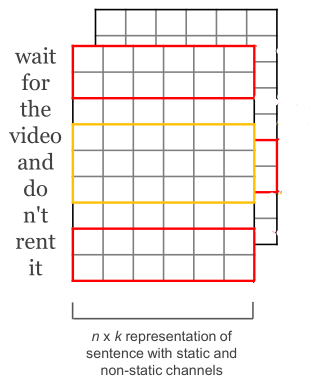10.2 Text-CNN的结构
（1）卷积层： 对某一句话对应的预训练的词向量矩阵维度为 $n*k$ ，设计一个过滤器窗口 $W$ ，其维度为 $h*k$，其中 $k$ 即为词向量的长度，$h$ 表示窗口所含的单词个数（Yoon 在实验中设置了 $h$ 的取值为2、3、4）。其次不断地滑动该窗口，每次滑动一个位置时，完成如下的计算：
$c_i=f(W·x_{i:i+h-1}+b)$
其中 $f$ 为非线性激活函数，x_{i:i+h-1}表示该句子中第 $i$ 到 $i+h-1$ 的单词组成的词向量矩阵，W·x_{i:i+h-1}表示两个矩阵的对应位乘积，$c_i$ 表示当前窗口位置的取值。
因此对于长度为 $n$ 的句子，维度为 $h*k$ 的过滤器窗口将可以产生 $n-h+1$ 个值组成的集合：
$c=\{c_1,c_2,...c_{n-h+1}\}$
（2）最大池化层：为了能够提取出其中最大的特征，Yoon对其进行max-over-time操作，即取出集合 $c$ 中的最大值 $\hat c=max{c}$。另外可以分析得到max-over-time操作还可以解决每句话长度不一致的问题。
Text-CNN的结构如图所示：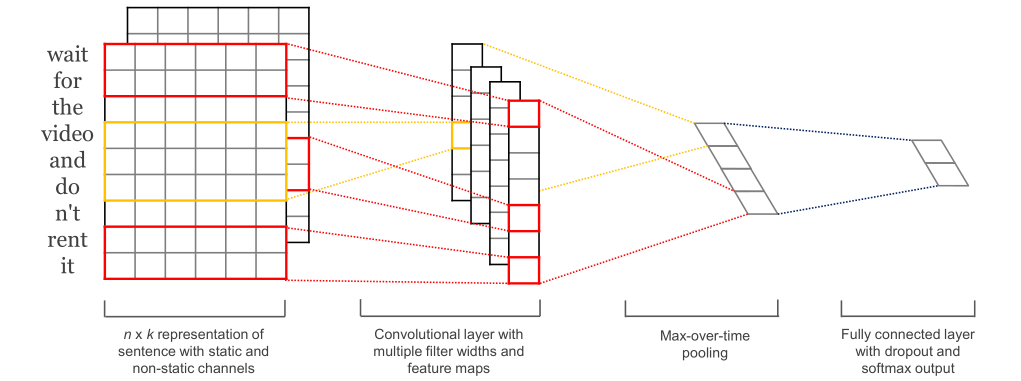（3）全相连层：对于 $m$ 个过滤器窗口，将产生 $m$ 个值组成的向量 $z=[\hat c_1,\hat c_2,...,\hat c_m]$，Text-CNN通过设置一个全相连层，将该向量映射为长度为 $l$ 的向量， $l$ 即为待预测的类的个数，设置softmax激活函数即可转换为各个类的概率值。
10.3 Text-CNN的训练
Text-CNN的前向传播即为上图所示流程。反向传播采用梯度下降法
（1）正则化防止过拟合：
对于 $m$ 个过滤器窗口产生的向量 $z=[\hat c_1,\hat c_2,...,\hat c_m]$ ，则输出值为 $y=w·z+b$，为了防止过拟合，采用 $l_2$ dropout权重衰减法，表达式为：
$y=w·(z\circ r)+b$
其中 $\circ$ 表示对应位置相乘， $r$ 表示以概率 $p$ 产生只含有0或1元素的矩阵， $p=0.5$ 则表示可能矩阵 $r$ 中有一半的元素为1 。
在测试环境，则为了限制 $l_2$ 范式的权重矩阵，设置 $||w||_2=s$ ，当$||w||_2>s$ 时进行梯度下降的调整。
Ps：$l_2$正则化权重衰减可参考：正则化方法：L1和L2 regularization、数据集扩增、dropout
（2）超参数设置：Yoon设置了一系列超参数如下：

超参数
取值

window（$h$值）
3/4/5

dropout（$p$值）
0.5

$l_2$（$s$值）
3

mini-batch
50

10.4 Text-CNN应用于关系抽取
Text-CNN在句子分类方面有不错的效果，而对于关系抽取问题，可以将其视为句子分类任务。
假设长度为 $n$ 具有 $m$ 个实体的句子 $x=\{x_1,x_2,...,x_n\}$ ，其中实体分别为 $e^{(i)}=\{x_{t_1}^{(i)},x_{t_2}^{(i)},...,x_{t_{k_i}}^{(i)}\}$，其中$i,t_{k_i}\in\mathbb[2,m]$， $k_i$ 表示第 $i$ 个实体的长度。该句子内所有实体将组成一个集合 $E_x=\{e^{(1)},e^{(2)},...,e^{(m)}\}$ 。任意取集合 $E_x$ 中的两个实体作为一个组合 $(e^{(a)},e^{(b)})$，其中 $(a ，并考察其是否具有关系，关系表示为 $r_{a,b}$ ，其取值为 $\{0,1,2,...,r\}$ ，$r$ 表示关系类标个数，即预设的试题关系的种类，每一个关系对应一个整数，0则表示没有关系。因此对于一个包含 $m$ 个实体 $E_x$ 的句子 $x$ 将产生 $h=C_m^2=\frac{m(m-1)}{2}$ 个关系组合 $R_x=\{r_{1,2},r_{1,3},...,r_{m-1,m}\}$。
是将一句话 $x$ 中不同组合的实体及其之间的所有单词组成一个新子句，并将其对应的word embeddings作为输入数据。子句 $x_{i,j}=\{e^{(i)},x_{t_{k_i}+1}^{(i)},...,x_{t_{k_j}-1}^{(i)},e^{(j)}\}$ ，其对应的关系类标为 $r_{i,j}$ ，因此对于 $x$ 将有 $h$ 个子句组成的样本集 $X=\{(x_{i,j},r_{i,j})|i 。
对于该样本，由于每个子句的长度不一致，因此需要进行填充0方式使各个子句长度一致，然后将其喂给Text-CNN。Text-CNN采用mini-batch法进行梯度下降，其训练过程可参考10.3节内容。
Ps：在诸多论文中都有表示，两个实体是否具有关系与其相对距x离有关系，而一般来说两者的距离超过25（即两个实体之间有超过25个单词），则两个实体具有关系的概率将会趋近于0，因此认为实体在一个句子内会产生关联，而不在同一个句子内认为不具有关联性，当然这种假设也存在一定的问题，但在实验中影响不大。

十一、基于依存关系模型的关系抽取
卷积神经网络可以很好的对实体与实体关系进行分类，而在自然语言处理中，通常会对句子进行句法分析，通过不同语言的语法规则建立起模型——依存关系。基于依存关系的关系抽取是该模型的一个应用方向，其主要是通过句法分析实现，而不是通过深度模型自动挖掘。本文虽然主要是以深度学习角度分析，但传统的“浅层态”模型也需要了解，以方便将其与深度模型进行整合。
11.1依存句法分析
基于依存关系模型的关系抽取也叫做开放式实体关系抽取，解决关系抽取的思路是对一个句子的词性进行预处理，例如对于一句话“马云在杭州创办了阿里巴巴”。不同于之前所讲的深度模型，词性分析则是对该句话中每一个词进行预先标注，例如“马云”、“杭州”和“阿里巴巴”被标记为名词，“在”和“了”被标记为介词，“创办”被标记为动词。所谓的依存关系则体现在不同词性的词之间存在着依存关系路径。“在”通常后面跟着地名，也就是名词，“创办”动词前通常为名词，而“在…创办了”便是一个依存关系。因此依存关系即为不同词性的词之间的关系结构，下标列出了关于中文的依存标注：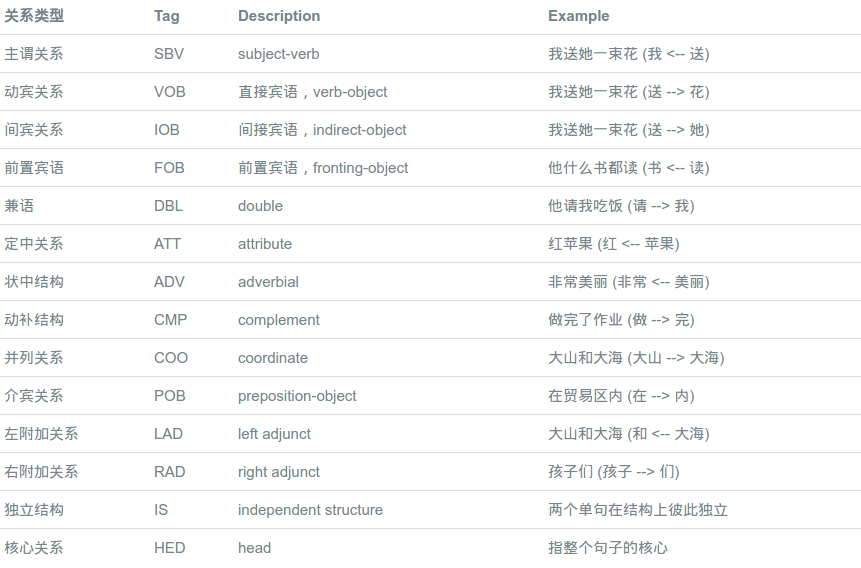例如对于下面一句话，句子开头设置一个“ROOT”作为开始，句子结束则为句号“。”，依存关系可以表示为下图：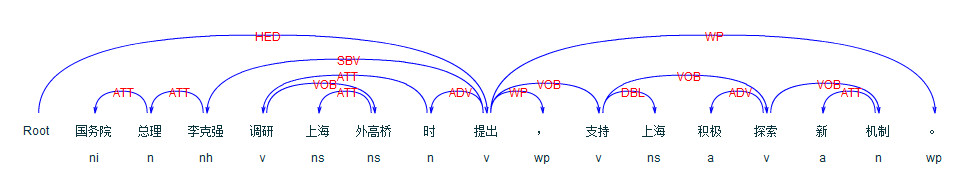在传统的实体识别中，是通过基于规则的词性分析实现的，最简单的是正则表达式匹配，其次是使用NLP词性标注工具。通常认为名词是实体，因此实体可以通过词性标注实现抽取。因为词性对每一个词进行了标注，自然根据语法规则可以构建起上图所示的依存关系。每一个词根据语法规则构建起一条关系路径。所有路径的最终起始点即为句子的核心（HED）。
11.2 依存句法分析实现关系抽取
对于关系抽取问题，基于依存关系的关系抽取模型中，关系词并非是预先设置的类别，而是存在于当前的句子中。例如“马云在杭州创办了阿里巴巴”，预定义的关系可能是“创始人”，而“创始人”一词在句子中不存在，但是句中存在一个与其相似的词“创办”。因此在句法分析中，能够提取出核心词“创办”，该词前面有一个名词“杭州”，而“杭州”前面有一个介词“在”，因此“在杭州”是一个介宾短语，依存路径被标记为POB，所以“杭州”不是“创办”的主语，自然是“马云”。“创办”一词后面是助词“了”可以省略，再往后则是名称“阿里巴巴”，因此“创办阿里巴巴”为动宾关系VOB，与上面的“探索机制”一样。因此可分析得到语义为“马云创办阿里巴巴”，核心词“创办”即为关系，“马云”和阿里巴巴则是两个实体。
因此基于依存关系的关系抽取算法步骤如下：
（1）获取句子 $x$；
（2）对句子 $x$ 进行词性标注；
（3）构建依存关系路径，并依据依存标注表对路径进行标注；
（4）提取核心词；
（5）构建起动宾结构，以核心词为关系寻找主语和宾语作为两个实体。
可以发现，基于依存关系不仅可以抽取关系，也可以提取出其对应的实体。因此包括基于深度学习模型在内，一种端到端的联合实体识别与关系抽取被提出。
十二、基于远程监督的关系抽取
参考博主写的博文：基于监督学习和远程监督的神经关系抽取
十三、注意力机制（Attention）
基于注意力机制的模型目前有许多，本文暂提供两种论文解读，详情博主两篇论文解读：
1.论文解读:Attention Is All You Need
2.论文解读：Attention-Based Bidirectional Long Short-Term Memory Networks for Relation Classification
十四、基于注意力机制的命名实体识别与关系抽取
应用注意力机制实现命名实体识别和关系抽取（也包括其他自然语言处理任务）成为常用之计，由于篇幅过大，本章节决定另开辟博文讲述。
十五、知识的存储——图形数据库
在通过一系列算法实现对非结构化数据进行命名实体识别和关系抽取之后，按照知识图谱中知识抽取的步骤将其存储在数据库中。通常使用的数据库为关系数据库，即满足第一和第二范式的“表格型”存储模型，但关系型数据库不能够很直接的处理具有关系类别的数据，即若表达不同数据之间的关系需要构建外键，并通过外连接方式进行关联，这对于百万级别数据来说，对查询速度以及后期的数据更新与维护都是不利的，因此需要一个能够体现不同实体关系的数据库。因此引入图数据库概念。
15.1 图数据库
图数据库包含的元素主要由结点、属性、关系，如图：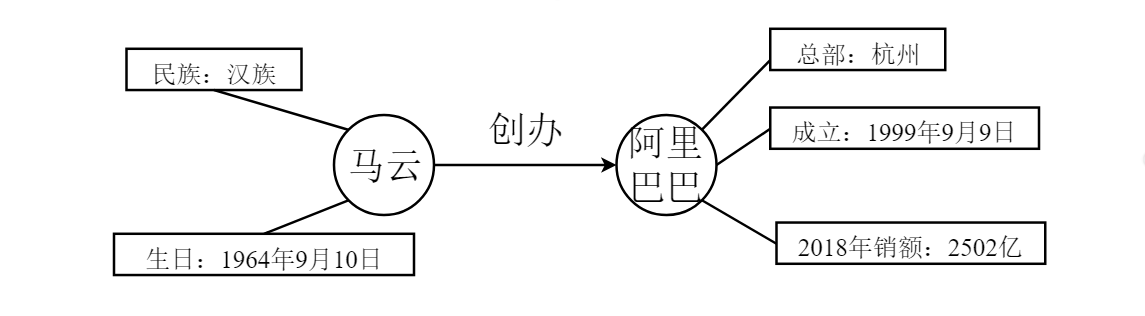“马云”和“阿里巴巴”为实体，对于图数据库中的结点，“马云” 与“阿里巴巴”二者实体之间有一个定向关系“创办”，对于于数据库中的关系，每个实体结点都有各自的属性，即图数据库中的属性，因此构建图数据库的关键三要素即为实体1，实体2和关系，即为三元组。
Ps：常用的图数据库有 Neo4j，NoSQL，Titan，OrientDB和 ArangoDB。关于图数据库的概念可参考：图数据库，数据库中的“黑科技”，初识图数据与图数据库。
15.2 Neo4j的简介
Neo4j是由java实现的开源NOSQL图数据库，数据库分为关系型和非关系型两种类型。其中非关系型又分为Graph(图形),Document(文档),Cloumn Family(列式)，以及Key-Value Store(KV)，这四种类型数据库分别使用不同的数据结构进行存储。因此它们所适用的场景也不尽相同。（引自Neo4j简介）
15.3 简单的CQL语句
在手动编辑Neo4j数据库时，需要编写CQL语句实现对数据库的操作。Java在实现对Neo4j时也需要编写CQL语句，因此在知识抽取后的保存工作，需要对CQL语句有所了解，下面是简单的CQL语句：
1、创建
（1）创建单个标签到结点
CREATE(结点名称:标签名)

（2）创建多个标签到结点：
CREATE(结点名称:标签名1:标签名2:....)

（3）创建结点和属性：
CREATE(结点名称:标签名{属性1名称:属性值1,属性2名称:属性值2,....})

（4）MERGE创建结点和属性（检测是否存在结点和属性一模一样的，若存在则不创建，不存在则创建）
MERGE(结点名称:标签名{属性1名称:属性值1,属性2名称:属性值2,....})

2、检索：
（1）检索一个结点
MATCH(结点名称:标签名) RETURN 结点名称

（2）检索一个结点的某个或多个属性
MATCH(结点名称:标签名) RETURN 结点名称.属性名称1,结点名称.属性名称2,...

（3）使用WHERE关键字搜索
MATCH(结点名称:标签名) WHERE 标签名.属性名 关系运算符（=>OR等） 数值 RETURN 结点名称.属性名称1,结点名称.属性名称2,...

Ps：其中WHERE 标签名.属性名 IS NOT NULL可以过滤掉不存在该属性值的结点
Ps：WHERE 标签名.属性名 IN [100,102] 表示筛选出该属性值介于100和102之间
（4）使用ORDER BY进行排序
MATCH(结点名称:标签名) RETURN 结点名称.属性名1，... ORDER BY 结点名称.属性名1

（DESC表示逆序，ASC表示正序）
（5）使用UNION进行联合查询（要求具有相同的列名称和数据类型，过滤掉重复数据）
MATCH(结点名称1.标签名)
RETURN 结点名称1.属性名1 as 属性名s1,结点名称1.属性名2 as 属性名s2,..
UNION
MATCH(结点名称2.标签名)
RETURN 结点名称2.属性名1 as 属性名s1,结点名称2.属性名2 as 属性名s2,..

Ps：若使用UNION ALL，则不过滤重复行，其他与UNION一致
（6）LIMIT限制返回记录个数
MATCH(结点名称:标签名称)
RETURN 结点名称.属性名1,...
LIMIT 5(限制最多返回5条数据)

（7）SKIP跳过前面的记录显示后面的
MATCH(结点名称:标签名称)
RETURN 结点名称.属性名1,...
SKIP 5(跳过前5条数据显示第6个及以后)

3、接点与关系
（1）添加新结点并为关系创建标签：
CREATE(结点1名称:标签名1)-[关系名称:关系标签名]->(结点2名称:标签名2)

（2）为现有的结点添加关系：
MATCH(结点1名称:标签名1)，(结点2名称:标签名2)
WHERE 条件
CREATE(结点1名称)-[关系名称:关系标签名]->(结点2名称)

（3）为现有结点添加/修改属性
MATCH(结点名称:标签名)
SET 结点名称.新属性名 = 值
RETURN 结点名称

4、删除
（1）删除结点：
MATCH(结点名称:标签名) DELETE 结点名称

（2）删除结点及关系
MATCH(结点1名称:标签名1)-[关系名称]->(结点2名称:标签名2)
DELETE 结点1名称,结点2名称,关系名称

（3）删除结点的属性
MATCH(结点名称:标签名)
REMOVE 结点名称.属性名,...
RETURN 结点名称

（4）删除结点的某个标签
MATCH(结点名称:标签名1)
REMOVE 结点名称:标签名2

十六、Tensorflow实现命名实体识别与关系抽取
本文以及详细的分析了实现命名实体识别与关系抽取的各种模型，本节将提供两个项目程序，一个是基于Bi-LSTM和CRF的命名实体识别，另一个是基于Bi-LSTM-LSTM和Text-CNN的端到端模型的实体与关系的联合抽取。
16.1 基于Bi-LSTM和CRF的命名实体识别
（1）main函数，主要解决控制台参数获取、数据获取以及调用模型：
import tensorflow as tf
import numpy as np
import os, argparse, time, random
from model import BiLSTM_CRF
from utils import str2bool, get_logger, get_entity
from data import read_corpus, read_dictionary, tag2label, random_embedding

## Tensorflow的Session运行环境
os.environ['CUDA_VISIBLE_DEVICES'] = '0'
os.environ['TF_CPP_MIN_LOG_LEVEL'] = '2'  # default: 0
config = tf.ConfigProto()
config.gpu_options.allow_growth = True
config.gpu_options.per_process_gpu_memory_fraction = 0.2  # need ~700MB GPU memory

## 控制台输入的超参数
parser = argparse.ArgumentParser(description='BiLSTM-CRF for Chinese NER task')
parser.add_argument('--train_data', type=str, default='data_path', help='train data source')
parser.add_argument('--test_data', type=str, default='data_path', help='test data source')
parser.add_argument('--batch_size', type=int, default=64, help='#sample of each minibatch')
parser.add_argument('--epoch', type=int, default=10, help='#epoch of training')
parser.add_argument('--hidden_dim', type=int, default=300, help='#dim of hidden state')
parser.add_argument('--optimizer', type=str, default='Adam', help='Adam/Adadelta/Adagrad/RMSProp/Momentum/SGD')
parser.add_argument('--CRF', type=str2bool, default=True, help='use CRF at the top layer. if False, use Softmax')
parser.add_argument('--lr', type=float, default=0.001, help='learning rate')
parser.add_argument('--clip', type=float, default=5.0, help='gradient clipping')
parser.add_argument('--dropout', type=float, default=0.5, help='dropout keep_prob')
parser.add_argument('--update_embedding', type=str2bool, default=True, help='update embedding during training')
parser.add_argument('--pretrain_embedding', type=str, default='random', help='use pretrained char embedding or init it randomly')
parser.add_argument('--embedding_dim', type=int, default=300, help='random init char embedding_dim')
parser.add_argument('--shuffle', type=str2bool, default=True, help='shuffle training data before each epoch')
parser.add_argument('--mode', type=str, default='demo', help='train/test/demo')
parser.add_argument('--demo_model', type=str, default='1521112368', help='model for test and demo')
args = parser.parse_args()

## 获取数据（word embeddings）
#word2id:为每一个不重复的字进行编号，其中UNK为最后一位
word2id = read_dictionary(os.path.join('.', args.train_data, 'word2id.pkl'))
print("\n========word2id=========\n",word2id)
if args.pretrain_embedding == 'random':
#随机生成词嵌入矩阵（一共3905个字，默认取300个特征，维度为3905*300）
embeddings = random_embedding(word2id, args.embedding_dim)
else:
embedding_path = 'pretrain_embedding.npy'
embeddings = np.array(np.load(embedding_path), dtype='float32')
print("\n=========embeddings==========\n",embeddings,"\ndim(embeddings)=",embeddings.shape)

## read corpus and get training data获取
if args.mode != 'demo':
train_path = os.path.join('.', args.train_data, 'train_data')
test_path = os.path.join('.', args.test_data, 'test_data')
train_data = read_corpus(train_path)#读取训练集
test_data = read_corpus(test_path); test_size = len(test_data)#读取测试集
#print("\n==========train_data================\n",train_data)
#print("\n==========test_data================\n",test_data)

## paths setting创建相应文件夹目录
paths = {}
# 时间戳就是一个时间点，一般就是为了在同步更新的情况下提高效率之用。
#就比如一个文件，如果他没有被更改，那么他的时间戳就不会改变，那么就没有必要写回，以提高效率，
#如果不论有没有被更改都重新写回的话，很显然效率会有所下降。
timestamp = str(int(time.time())) if args.mode == 'train' else args.demo_model
#输出路径output_path路径设置为data_path_save下的具体时间名字为文件名
output_path = os.path.join('.', args.train_data+"_save", timestamp)
if not os.path.exists(output_path): os.makedirs(output_path)

summary_path = os.path.join(output_path, "summaries")
paths['summary_path'] = summary_path
if not os.path.exists(summary_path): os.makedirs(summary_path)
model_path = os.path.join(output_path, "checkpoints/")
if not os.path.exists(model_path): os.makedirs(model_path)
ckpt_prefix = os.path.join(model_path, "model")
paths['model_path'] = ckpt_prefix
result_path = os.path.join(output_path, "results")
paths['result_path'] = result_path
if not os.path.exists(result_path): os.makedirs(result_path)
log_path = os.path.join(result_path, "log.txt")
paths['log_path'] = log_path
get_logger(log_path).info(str(args))

## 调用模型进行训练
if args.mode == 'train':
#创建对象model
model = BiLSTM_CRF(args, embeddings, tag2label, word2id, paths, config=config)
#创建结点，
model.build_graph()

## hyperparameters-tuning, split train/dev
# dev_data = train_data[:5000]; dev_size = len(dev_data)
# train_data = train_data[5000:]; train_size = len(train_data)
# print("train data: {0}\ndev data: {1}".format(train_size, dev_size))
# model.train(train=train_data, dev=dev_data)

## train model on the whole training data
print("train data: {}".format(len(train_data)))
model.train(train=train_data, dev=test_data)  # use test_data as the dev_data to see overfitting phenomena

## 调用模型进行测试
elif args.mode == 'test':
ckpt_file = tf.train.latest_checkpoint(model_path)
print(ckpt_file)
paths['model_path'] = ckpt_file
model = BiLSTM_CRF(args, embeddings, tag2label, word2id, paths, config=config)
model.build_graph()
print("test data: {}".format(test_size))
model.test(test_data)

## 根据训练并测试好的模型进行应用
elif args.mode == 'demo':
ckpt_file = tf.train.latest_checkpoint(model_path)
print(ckpt_file)
paths['model_path'] = ckpt_file
model = BiLSTM_CRF(args, embeddings, tag2label, word2id, paths, config=config)
model.build_graph()
saver = tf.train.Saver()
with tf.Session(config=config) as sess:
saver.restore(sess, ckpt_file)
while(1):
print('Please input your sentence:')
demo_sent = input()
if demo_sent == '' or demo_sent.isspace():
print('bye!')
break
else:
demo_sent = list(demo_sent.strip())
demo_data = [(demo_sent, ['O'] * len(demo_sent))]
tag = model.demo_one(sess, demo_data)
PER, LOC, ORG = get_entity(tag, demo_sent)
print('PER: {}\nLOC: {}\nORG: {}'.format(PER, LOC, ORG))

（2）数据处理module，处理数据，包括对数据进行编号，词向量获取以及标注信息的处理等：
import sys, pickle, os, random
import numpy as np

## tags, BIO
tag2label = {"O": 0,
"B-PER": 1, "I-PER": 2,
"B-LOC": 3, "I-LOC": 4,
"B-ORG": 5, "I-ORG": 6
}

#输入train_data文件的路径，读取训练集的语料，输出train_data
def read_corpus(corpus_path):
"""
read corpus and return the list of samples
:param corpus_path:
:return: data
"""
data = []
with open(corpus_path, encoding='utf-8') as fr:
lines = fr.readlines()
sent_, tag_ = [], []
for line in lines:
if line != '\n':
[char, label] = line.strip().split()
sent_.append(char)
tag_.append(label)
else:
data.append((sent_, tag_))
sent_, tag_ = [], []
return data

#生成word2id序列化文件
def vocab_build(vocab_path, corpus_path, min_count):
"""
#建立词汇表
:param vocab_path:
:param corpus_path:
:param min_count:
:return:
"""
#读取数据（训练集或测试集）
#data格式：[(字,标签),...]
data = read_corpus(corpus_path)
word2id = {}
for sent_, tag_ in data:
for word in sent_:
if word.isdigit():
word = '<NUM>'
elif ('\u0041' <= word <='\u005a') or ('\u0061' <= word <='\u007a'):
word = '<ENG>'
if word not in word2id:
word2id[word] = [len(word2id)+1, 1]
else:
word2id[word] += 1
low_freq_words = []
for word, [word_id, word_freq] in word2id.items():
if word_freq < min_count and word != '<NUM>' and word != '<ENG>':
low_freq_words.append(word)
for word in low_freq_words:
del word2id[word]
new_id = 1
for word in word2id.keys():
word2id[word] = new_id
new_id += 1
word2id['<UNK>'] = new_id
word2id['<PAD>'] = 0
print(len(word2id))
#将任意对象进行序列化保存
with open(vocab_path, 'wb') as fw:
pickle.dump(word2id, fw)

#将句子中每一个字转换为id编号，例如['我','爱','中','国'] ==> ['453','7','3204','550']
def sentence2id(sent, word2id):
"""
:param sent:源句子
:param word2id:对应的转换表
:return:
"""
sentence_id = []
for word in sent:
if word.isdigit():
word = '<NUM>'
elif ('\u0041' <= word <= '\u005a') or ('\u0061' <= word <= '\u007a'):
word = '<ENG>'
if word not in word2id:
word = '<UNK>'
sentence_id.append(word2id[word])
return sentence_id

#读取word2id文件
def read_dictionary(vocab_path):
"""
:param vocab_path:
:return:
"""
vocab_path = os.path.join(vocab_path)
#反序列化
with open(vocab_path, 'rb') as fr:
word2id = pickle.load(fr)
print('vocab_size:', len(word2id))
return word2id

#随机嵌入
def random_embedding(vocab, embedding_dim):
"""
:param vocab:
:param embedding_dim:
:return:
"""
embedding_mat = np.random.uniform(-0.25, 0.25, (len(vocab), embedding_dim))
embedding_mat = np.float32(embedding_mat)
return embedding_mat

def pad_sequences(sequences, pad_mark=0):
"""
:param sequences:
:param pad_mark:
:return:
"""
max_len = max(map(lambda x : len(x), sequences))
seq_list, seq_len_list = [], []
for seq in sequences:
seq = list(seq)
seq_ = seq[:max_len] + [pad_mark] * max(max_len - len(seq), 0)
seq_list.append(seq_)
seq_len_list.append(min(len(seq), max_len))
return seq_list, seq_len_list

def batch_yield(data, batch_size, vocab, tag2label, shuffle=False):
"""
:param data:
:param batch_size:
:param vocab:
:param tag2label:
:param shuffle:随机对列表data进行排序
:return:
"""
#如果参数shuffle为true，则对data列表进行随机排序
if shuffle:
random.shuffle(data)
seqs, labels = [], []
for (sent_, tag_) in data:
#将句子转换为编号组成的数字序列
sent_ = sentence2id(sent_, vocab)
#将标签序列转换为数字序列
label_ = [tag2label[tag] for tag in tag_]
#一个句子就是一个样本，当句子数量等于预设的一批训练集数量，便输出该样本
if len(seqs) == batch_size:
yield seqs, labels
seqs, labels = [], []
seqs.append(sent_)
labels.append(label_)

if len(seqs) != 0:
yield seqs, labels

（3）二进制读写
import os
def conlleval(label_predict, label_path, metric_path):
"""
:param label_predict:
:param label_path:
:param metric_path:
:return:
"""
eval_perl = "./conlleval_rev.pl"
with open(label_path, "w") as fw:
line = []
for sent_result in label_predict:
for char, tag, tag_ in sent_result:
tag = '0' if tag == 'O' else tag
char = char.encode("utf-8")
line.append("{} {} {}\n".format(char, tag, tag_))
line.append("\n")
fw.writelines(line)
os.system("perl {} < {} > {}".format(eval_perl, label_path, metric_path))
with open(metric_path) as fr:
metrics = [line.strip() for line in fr]
return metrics

（4）字符串处理，对数据类标转换，对已生成的标注序列进行实体提取：
import logging, sys, argparse

#将字符串转换为布尔型
def str2bool(v):
# copy from StackOverflow
if v.lower() in ('yes', 'true', 't', 'y', '1'):
return True
elif v.lower() in ('no', 'false', 'f', 'n', '0'):
return False
else:
raise argparse.ArgumentTypeError('Boolean value expected.')

#获得实体（将标签序列找出相应的标签组合并返回对应的文字）
def get_entity(tag_seq, char_seq):
PER = get_PER_entity(tag_seq, char_seq)
LOC = get_LOC_entity(tag_seq, char_seq)
ORG = get_ORG_entity(tag_seq, char_seq)
return PER, LOC, ORG

def get_PER_entity(tag_seq, char_seq):
length = len(char_seq)
PER = []
for i, (char, tag) in enumerate(zip(char_seq, tag_seq)):
if tag == 'B-PER':
if 'per' in locals().keys():
PER.append(per)
del per
per = char
if i+1 == length:
PER.append(per)
if tag == 'I-PER':
per += char
if i+1 == length:
PER.append(per)
if tag not in ['I-PER', 'B-PER']:
if 'per' in locals().keys():
PER.append(per)
del per
continue
return PER

def get_LOC_entity(tag_seq, char_seq):
length = len(char_seq)
LOC = []
for i, (char, tag) in enumerate(zip(char_seq, tag_seq)):
if tag == 'B-LOC':
if 'loc' in locals().keys():
LOC.append(loc)
del loc
loc = char
if i+1 == length:
LOC.append(loc)
if tag == 'I-LOC':
loc += char
if i+1 == length:
LOC.append(loc)
if tag not in ['I-LOC', 'B-LOC']:
if 'loc' in locals().keys():
LOC.append(loc)
del loc
continue
return LOC

def get_ORG_entity(tag_seq, char_seq):
length = len(char_seq)
ORG = []
for i, (char, tag) in enumerate(zip(char_seq, tag_seq)):
if tag == 'B-ORG':
if 'org' in locals().keys():
ORG.append(org)
del org
org = char
if i+1 == length:
ORG.append(org)
if tag == 'I-ORG':
org += char
if i+1 == length:
ORG.append(org)
if tag not in ['I-ORG', 'B-ORG']:
if 'org' in locals().keys():
ORG.append(org)
del org
continue
return ORG

def get_logger(filename):
logger = logging.getLogger('logger')
logger.setLevel(logging.DEBUG)
logging.basicConfig(format='%(message)s', level=logging.DEBUG)
handler = logging.FileHandler(filename)
handler.setLevel(logging.DEBUG)
handler.setFormatter(logging.Formatter('%(asctime)s:%(levelname)s: %(message)s'))
logging.getLogger().addHandler(handler)
return logger

（5）Bi-LSTM+CRF模型：
import numpy as np
import os, time, sys
import tensorflow as tf
from tensorflow.contrib.rnn import LSTMCell
from tensorflow.contrib.crf import crf_log_likelihood
from tensorflow.contrib.crf import viterbi_decode
from data import pad_sequences, batch_yield
from utils import get_logger
from eval import conlleval

#batch_size：批大小，一次训练的样本数量
#epoch：使用全部训练样本训练的次数
#hidden_dim：隐藏维度
#embeddinds:词嵌入矩阵（字数量*特征数量）
class BiLSTM_CRF(object):
def __init__(self, args, embeddings, tag2label, vocab, paths, config):
self.batch_size = args.batch_size
self.epoch_num = args.epoch
self.hidden_dim = args.hidden_dim
self.embeddings = embeddings
self.CRF = args.CRF
self.update_embedding = args.update_embedding
self.dropout_keep_prob = args.dropout
self.optimizer = args.optimizer
self.lr = args.lr
self.clip_grad = args.clip
self.tag2label = tag2label
self.num_tags = len(tag2label)
self.vocab = vocab
self.shuffle = args.shuffle
self.model_path = paths['model_path']
self.summary_path = paths['summary_path']
self.logger = get_logger(paths['log_path'])
self.result_path = paths['result_path']
self.config = config

#创建结点
def build_graph(self):
self.add_placeholders()
self.lookup_layer_op()
self.biLSTM_layer_op()
self.softmax_pred_op()
self.loss_op()
self.trainstep_op()
self.init_op()

#placeholder相当于定义了一个位置，这个位置中的数据在程序运行时再指定
def add_placeholders(self):
self.word_ids = tf.placeholder(tf.int32, shape=[None, None], name="word_ids")
self.labels = tf.placeholder(tf.int32, shape=[None, None], name="labels")
self.sequence_lengths = tf.placeholder(tf.int32, shape=[None], name="sequence_lengths")
self.dropout_pl = tf.placeholder(dtype=tf.float32, shape=[], name="dropout")
self.lr_pl = tf.placeholder(dtype=tf.float32, shape=[], name="lr")

def lookup_layer_op(self):
#新建变量
with tf.variable_scope("words"):
_word_embeddings = tf.Variable(self.embeddings,
dtype=tf.float32,
trainable=self.update_embedding,
name="_word_embeddings")
#寻找_word_embeddings矩阵中分别为words_ids中元素作为下标的值
#提取出该句子每个字对应的向量并组合起来
word_embeddings = tf.nn.embedding_lookup(params=_word_embeddings,
ids=self.word_ids,
name="word_embeddings")
#dropout函数是为了防止在训练中过拟合的操作，将训练输出按一定规则进行变换
self.word_embeddings =  tf.nn.dropout(word_embeddings, self.dropout_pl)
print("========word_embeddings=============\n",word_embeddings)

#双向LSTM网络层输出
def biLSTM_layer_op(self):
with tf.variable_scope("bi-lstm"):
cell_fw = LSTMCell(self.hidden_dim)#前向cell
cell_bw = LSTMCell(self.hidden_dim)#反向cell
#(output_fw_seq, output_bw_seq)是一个包含前向cell输出tensor和后向cell输出tensor组成的元组，_为包含了前向和后向最后的隐藏状态的组成的元组
(output_fw_seq, output_bw_seq), _ = tf.nn.bidirectional_dynamic_rnn(
cell_fw=cell_fw,
cell_bw=cell_bw,
inputs=self.word_embeddings,
sequence_length=self.sequence_lengths,
dtype=tf.float32)
output = tf.concat([output_fw_seq, output_bw_seq], axis=-1)
output = tf.nn.dropout(output, self.dropout_pl)
with tf.variable_scope("proj"):
#权值矩阵
W = tf.get_variable(name="W",
shape=[2 * self.hidden_dim, self.num_tags],
initializer=tf.contrib.layers.xavier_initializer(),
dtype=tf.float32)
#阈值向量
b = tf.get_variable(name="b",
shape=[self.num_tags],
initializer=tf.zeros_initializer(),
dtype=tf.float32)
s = tf.shape(output)
output = tf.reshape(output, [-1, 2*self.hidden_dim])
#tf.matmul矩阵乘法
pred = tf.matmul(output, W) + b
self.logits = tf.reshape(pred, [-1, s, self.num_tags])

#损失函数
def loss_op(self):
#使用CRF的最大似然估计
if self.CRF:
#crf_log_likelihood作为损失函数
#inputs：unary potentials,就是每个标签的预测概率值
#tag_indices，这个就是真实的标签序列了
#sequence_lengths,一个样本真实的序列长度，为了对齐长度会做些padding，但是可以把真实的长度放到这个参数里
#transition_params,转移概率，可以没有，没有的话这个函数也会算出来
#输出：log_likelihood:标量;transition_params,转移概率，如果输入没输，它就自己算个给返回
#self.logits为双向LSTM的输出
log_likelihood, self.transition_params = crf_log_likelihood(inputs=self.logits,
tag_indices=self.labels,
sequence_lengths=self.sequence_lengths)
#tf.reduce_mean默认对log_likelihood所有元素求平均
self.loss = -tf.reduce_mean(log_likelihood)
else:
#交差信息熵
losses = tf.nn.sparse_softmax_cross_entropy_with_logits(logits=self.logits,
labels=self.labels)
mask = tf.sequence_mask(self.sequence_lengths)
losses = tf.boolean_mask(losses, mask)
self.loss = tf.reduce_mean(losses)
tf.summary.scalar("loss", self.loss)

def softmax_pred_op(self):
if not self.CRF:
self.labels_softmax_ = tf.argmax(self.logits, axis=-1)
self.labels_softmax_ = tf.cast(self.labels_softmax_, tf.int32)

def trainstep_op(self):
with tf.variable_scope("train_step"):
#global_step:Optional Variable to increment by one after the variables have been updated
self.global_step = tf.Variable(0, name="global_step", trainable=False)
#选择优化算法
if self.optimizer == 'Adam':
optim = tf.train.AdamOptimizer(learning_rate=self.lr_pl)
elif self.optimizer == 'Adadelta':
optim = tf.train.AdadeltaOptimizer(learning_rate=self.lr_pl)
elif self.optimizer == 'Adagrad':
optim = tf.train.AdagradOptimizer(learning_rate=self.lr_pl)
elif self.optimizer == 'RMSProp':
optim = tf.train.RMSPropOptimizer(learning_rate=self.lr_pl)
elif self.optimizer == 'Momentum':
optim = tf.train.MomentumOptimizer(learning_rate=self.lr_pl, momentum=0.9)
elif self.optimizer == 'SGD':
optim = tf.train.GradientDescentOptimizer(learning_rate=self.lr_pl)
else:
optim = tf.train.GradientDescentOptimizer(learning_rate=self.lr_pl)
#根据优化算法模型计算损失函数梯度，返回梯度和变量列表
grads_and_vars = optim.compute_gradients(self.loss)
#tf.clip_by_value(A, min, max)指将列表A中元素压缩在min和max之间，大于max或小于min的值改成max和min
#梯度修剪
grads_and_vars_clip = [[tf.clip_by_value(g, -self.clip_grad, self.clip_grad), v] for g, v in grads_and_vars]
#grads_and_vars_clip: compute_gradients()函数返回的(gradient, variable)对的列表并修剪后的
#global_step:Optional Variable to increment by one after the variables have been updated
self.train_op = optim.apply_gradients(grads_and_vars_clip, global_step=self.global_step)

def init_op(self):
self.init_op = tf.global_variables_initializer()
#显示训练过程中的信息
def add_summary(self, sess):
"""
:param sess:
:return:
"""
self.merged = tf.summary.merge_all()
#指定一个文件用来保存图。
self.file_writer = tf.summary.FileWriter(self.summary_path, sess.graph)

def train(self, train, dev):
"""
:param train:
:param dev:
:return:
"""
#创建保存模型对象
saver = tf.train.Saver(tf.global_variables())
with tf.Session(config=self.config) as sess:
sess.run(self.init_op)
self.add_summary(sess)
#循环训练epoch_num次
for epoch in range(self.epoch_num):
self.run_one_epoch(sess, train, dev, self.tag2label, epoch, saver)

def test(self, test):
saver = tf.train.Saver()
with tf.Session(config=self.config) as sess:
self.logger.info('=========== testing ===========')
saver.restore(sess, self.model_path)
label_list, seq_len_list = self.dev_one_epoch(sess, test)
self.evaluate(label_list, seq_len_list, test)

#demo
def demo_one(self, sess, sent):
"""
:param sess:
:param sent:
:return:
"""
label_list = []
#随机将句子分批次，并遍历这些批次，对每一批数据进行预测
for seqs, labels in batch_yield(sent, self.batch_size, self.vocab, self.tag2label, shuffle=False):
#预测该批样本，并返回相应的标签数字序列
label_list_, _ = self.predict_one_batch(sess, seqs)
label_list.extend(label_list_)
label2tag = {}
for tag, label in self.tag2label.items():
label2tag[label] = tag if label != 0 else label
#根据标签对照表将数字序列转换为文字标签序列
tag = [label2tag[label] for label in label_list]
print('===mode.demo_one:','label_list=',label_list,',label2tag=',label2tag,',tag=',tag)
return tag

#训练一次
def run_one_epoch(self, sess, train, dev, tag2label, epoch, saver):
"""
:param sess:
:param train:训练集
:param dev:验证集
:param tag2label:标签转换字典
:param epoch:当前训练的轮数
:param saver:保存的模型
:return:
"""
#训练批次数
num_batches = (len(train) + self.batch_size - 1) // self.batch_size
start_time = time.strftime("%Y-%m-%d %H:%M:%S", time.localtime())
#随机为每一批分配数据
batches = batch_yield(train, self.batch_size, self.vocab, self.tag2label, shuffle=self.shuffle)
#训练每一批训练集
for step, (seqs, labels) in enumerate(batches):
sys.stdout.write(' processing: {} batch / {} batches.'.format(step + 1, num_batches) + '\r')
step_num = epoch * num_batches + step + 1
feed_dict, _ = self.get_feed_dict(seqs, labels, self.lr, self.dropout_keep_prob)
_, loss_train, summary, step_num_ = sess.run([self.train_op, self.loss, self.merged, self.global_step],
feed_dict=feed_dict)
if step + 1 == 1 or (step + 1) % 300 == 0 or step + 1 == num_batches:
self.logger.info(
'{} epoch {}, step {}, loss: {:.4}, global_step: {}'.format(start_time, epoch + 1, step + 1,
loss_train, step_num))
self.file_writer.add_summary(summary, step_num)
#保存模型
if step + 1 == num_batches:
#保存模型数据
#第一个参数sess,这个就不用说了。第二个参数设定保存的路径和名字，第三个参数将训练的次数作为后缀加入到模型名字中。
saver.save(sess, self.model_path, global_step=step_num)
self.logger.info('===========validation / test===========')
label_list_dev, seq_len_list_dev = self.dev_one_epoch(sess, dev)
#模型评估
self.evaluate(label_list_dev, seq_len_list_dev, dev, epoch)

def get_feed_dict(self, seqs, labels=None, lr=None, dropout=None):
"""
:param seqs:句子数字序列
:param labels:对应句子的标签数字序列
:param lr:
:param dropout:
:return: feed_dict
"""
#获取填充0后的句子序列样本（使得每个句子填充0后长度一致），同时记录每个句子实际长度
#例如：s = [['3','1','6','34','66','8'],['3','1','34','66','8'],['3','1','6','34']]
#返回为[['3', '1', '6', '34', '66', '8'], ['3', '1', '34', '66', '8', 0], ['3', '1', '6', '34', 0, 0]],长度为 [6, 5, 4]
word_ids, seq_len_list = pad_sequences(seqs, pad_mark=0)
feed_dict = {self.word_ids: word_ids,
self.sequence_lengths: seq_len_list}
if labels is not None:
#为标签序列填充0并返回每个句子标签的实际长度
labels_, _ = pad_sequences(labels, pad_mark=0)
feed_dict[self.labels] = labels_
if lr is not None:
feed_dict[self.lr_pl] = lr
if dropout is not None:
feed_dict[self.dropout_pl] = dropout
return feed_dict, seq_len_list

def dev_one_epoch(self, sess, dev):
"""
:param sess:
:param dev:
:return:
"""
label_list, seq_len_list = [], []
for seqs, labels in batch_yield(dev, self.batch_size, self.vocab, self.tag2label, shuffle=False):
label_list_, seq_len_list_ = self.predict_one_batch(sess, seqs)
label_list.extend(label_list_)
seq_len_list.extend(seq_len_list_)
return label_list, seq_len_list

#预测一批数据集
def predict_one_batch(self, sess, seqs):
"""
:param sess:
:param seqs:
:return: label_list
seq_len_list
"""
#将样本进行整理（填充0方式使得每句话长度一样，并返回每句话实际长度）
feed_dict, seq_len_list = self.get_feed_dict(seqs, dropout=1.0)
#若使用CRF
if self.CRF:
logits, transition_params = sess.run([self.logits, self.transition_params],
feed_dict=feed_dict)
label_list = []
for logit, seq_len in zip(logits, seq_len_list):
viterbi_seq, _ = viterbi_decode(logit[:seq_len], transition_params)
label_list.append(viterbi_seq)
return label_list, seq_len_list
else:
label_list = sess.run(self.labels_softmax_, feed_dict=feed_dict)
return label_list, seq_len_list

def evaluate(self, label_list, seq_len_list, data, epoch=None):
"""
:param label_list:
:param seq_len_list:
:param data:
:param epoch:
:return:
"""
label2tag = {}
for tag, label in self.tag2label.items():
label2tag[label] = tag if label != 0 else label
model_predict = []
for label_, (sent, tag) in zip(label_list, data):
tag_ = [label2tag[label__] for label__ in label_]
sent_res = []
if  len(label_) != len(sent):
print(sent)
print(len(label_))
print(tag)
for i in range(len(sent)):
sent_res.append([sent[i], tag[i], tag_[i]])
model_predict.append(sent_res)
epoch_num = str(epoch+1) if epoch != None else 'test'
label_path = os.path.join(self.result_path, 'label_' + epoch_num)
metric_path = os.path.join(self.result_path, 'result_metric_' + epoch_num)
for _ in conlleval(model_predict, label_path, metric_path):
self.logger.info(_)

十七、推荐阅读书籍
非常能够感谢你能够通读整片文章，本人将针对基于深度学习的命名实体识别与关系抽取为主题，推荐学习文献或书籍。
17.1 推荐的书籍
（1）《统计学习方法》（李航）
这本书是主要针对自然语言处理领域编写的统计学习方法。统计学习方法又叫做统计机器学习。这本书很细致的讲解了几大典型的机器学习算法，同时针对每一种算法的模型进行了相关推导。另外书中还细讲了图概率模型、隐马尔可夫模型和条件随机场，这对于理解命名实体识别非常有利。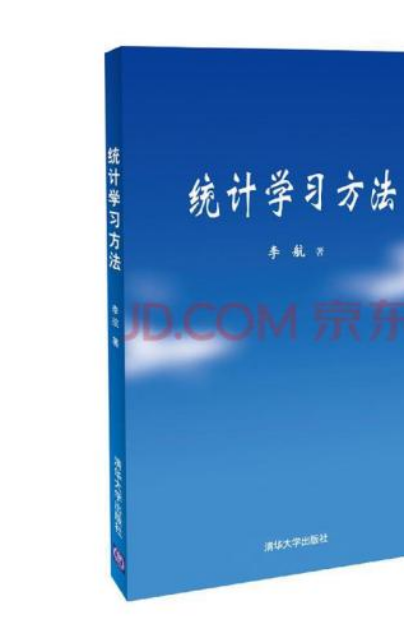（2）《机器学习》（周志华）
南京大学人工智能实验室的主任周志华在机器学习和大数据挖掘领域内是国内外知名的“大佬”，他出版的《机器学习》西瓜书已经成为IT领域内的畅销书。本人阅读了该书，认为该书对数学理论要求非常高，因此这本书需要在对基本的机器学习知识有所了解之后才适合读。因此较为合理的应先阅读《统计学习方法》后再阅读《机器学习》。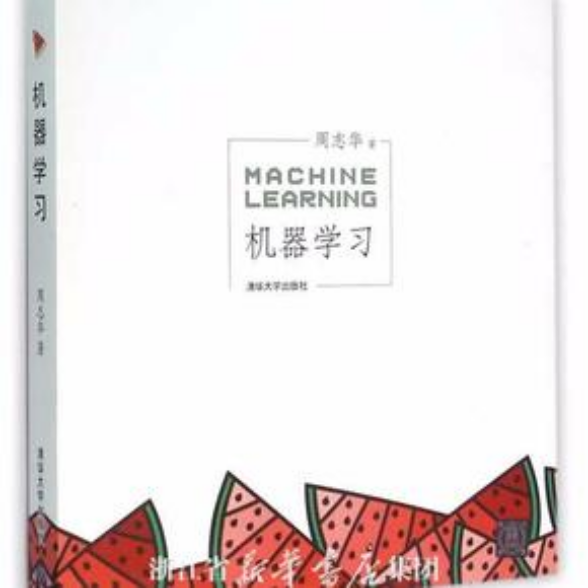（3）《深度学习》（[美] 伊恩·古德费洛）
《深度学习》是美国数学家伊恩·古德费洛所编写，由人民邮电出版社出版。该书主要以深度学习为主，再简单介绍了机器学习的知识后，对深度学习进行了多角度的分析，并介绍了多层感知机BP神经网络、循环神经网络、卷积神经网络、计算图等内容，是深度学习领域内非常权威的书籍。因此在学习完基本的机器学习内容之后，学习深度学习是必要的。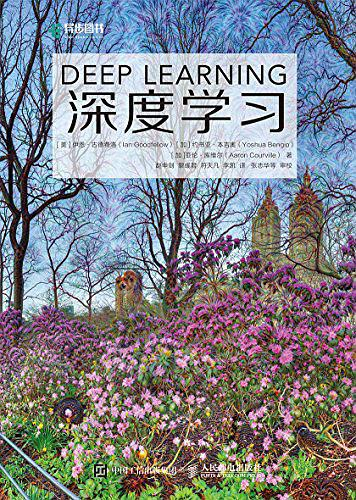（4）另外推荐几本数学类书籍，对数学方面薄弱的或者没有学习的读者提供学习的方向：
《微积分》、《线性代数·同济版》、《矩阵论·华科版》、《最优化方法及其应用》、《最优控制》等。
十八、项目实例1
项目名称：面向智慧农业的知识图谱及其应用系统
研究课题：上海市《农业信息服务平台及农业大数据综合利用研究》之《上海农业农村大数据共享服务平台建设和应用》
项目团队：华东师范大学数据科学与工程学院
项目开源：https://github.com/qq547276542/Agriculture_KnowledgeGraph
运行界面：项目部署可参考GitHub上的README。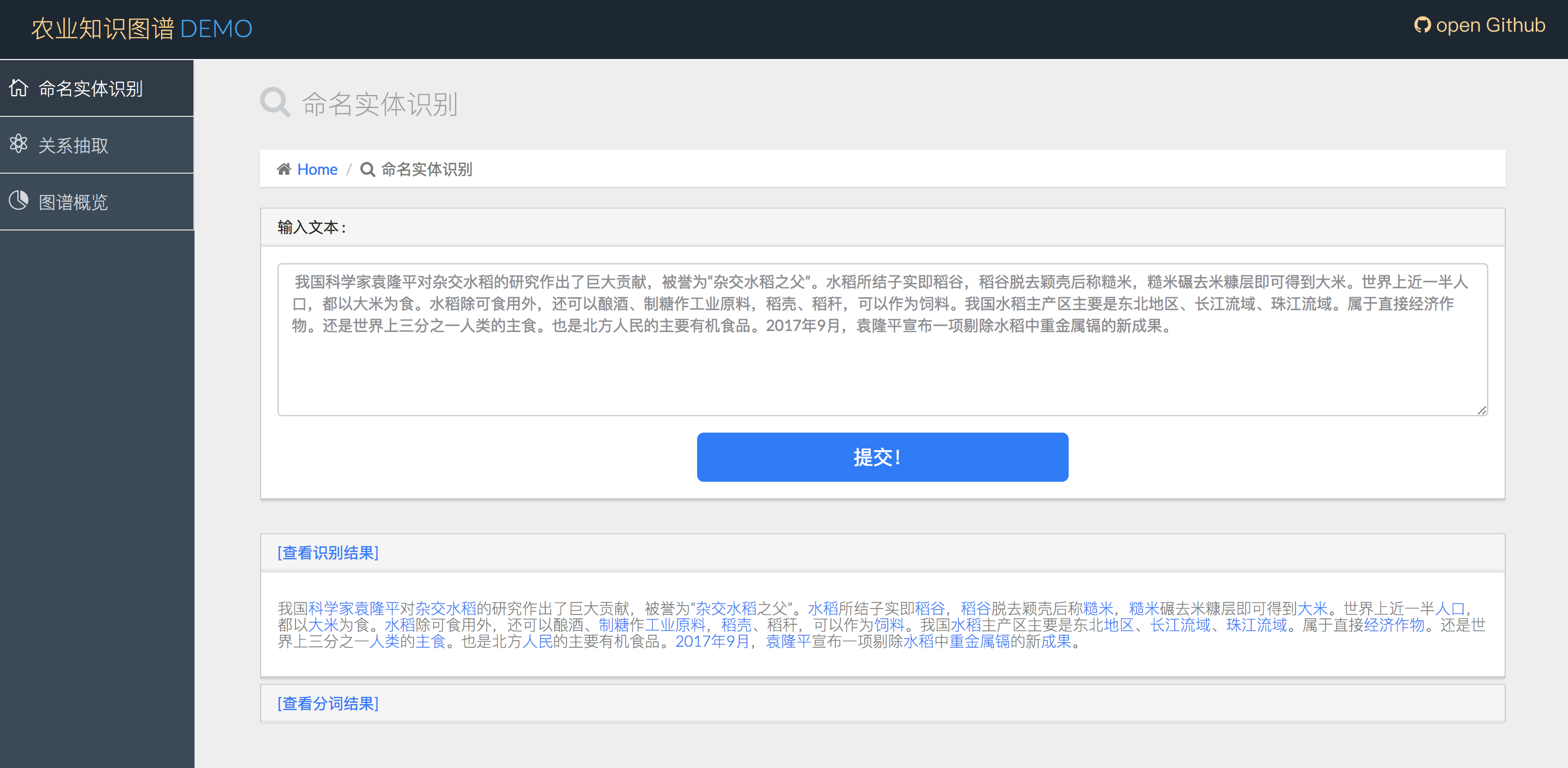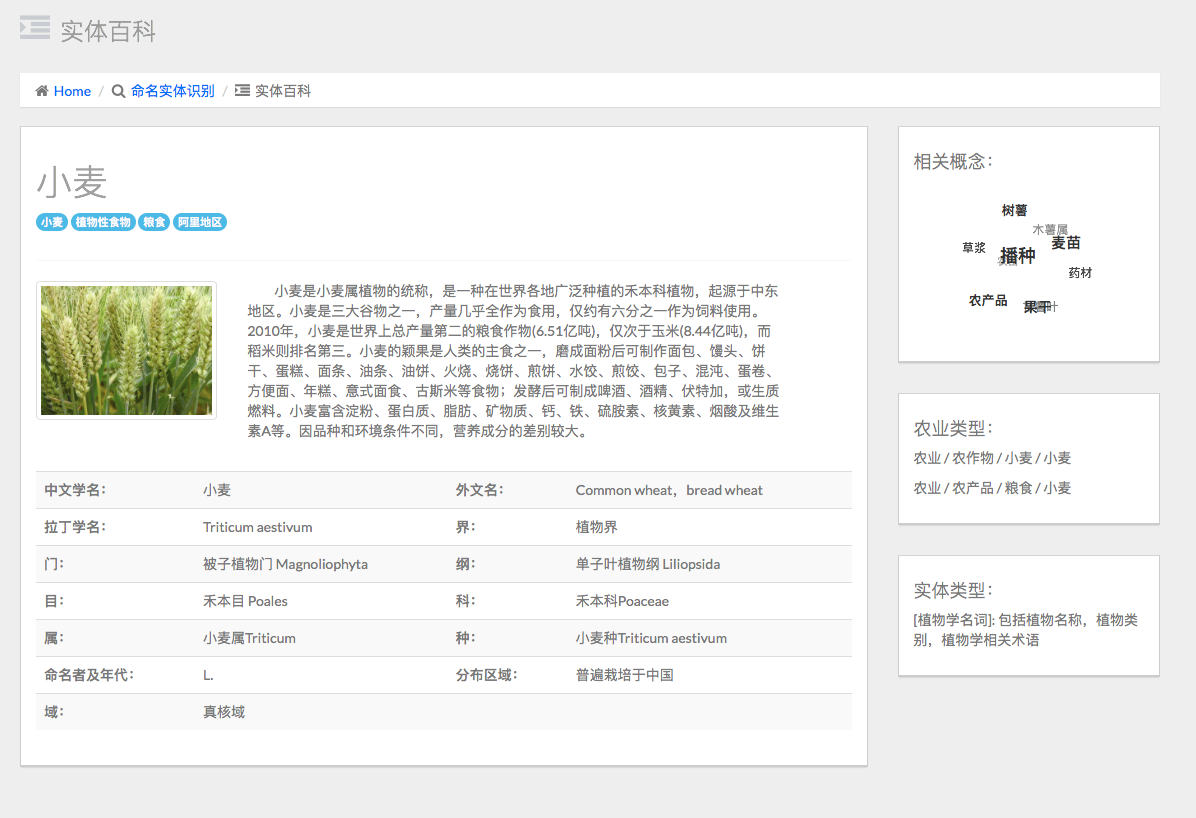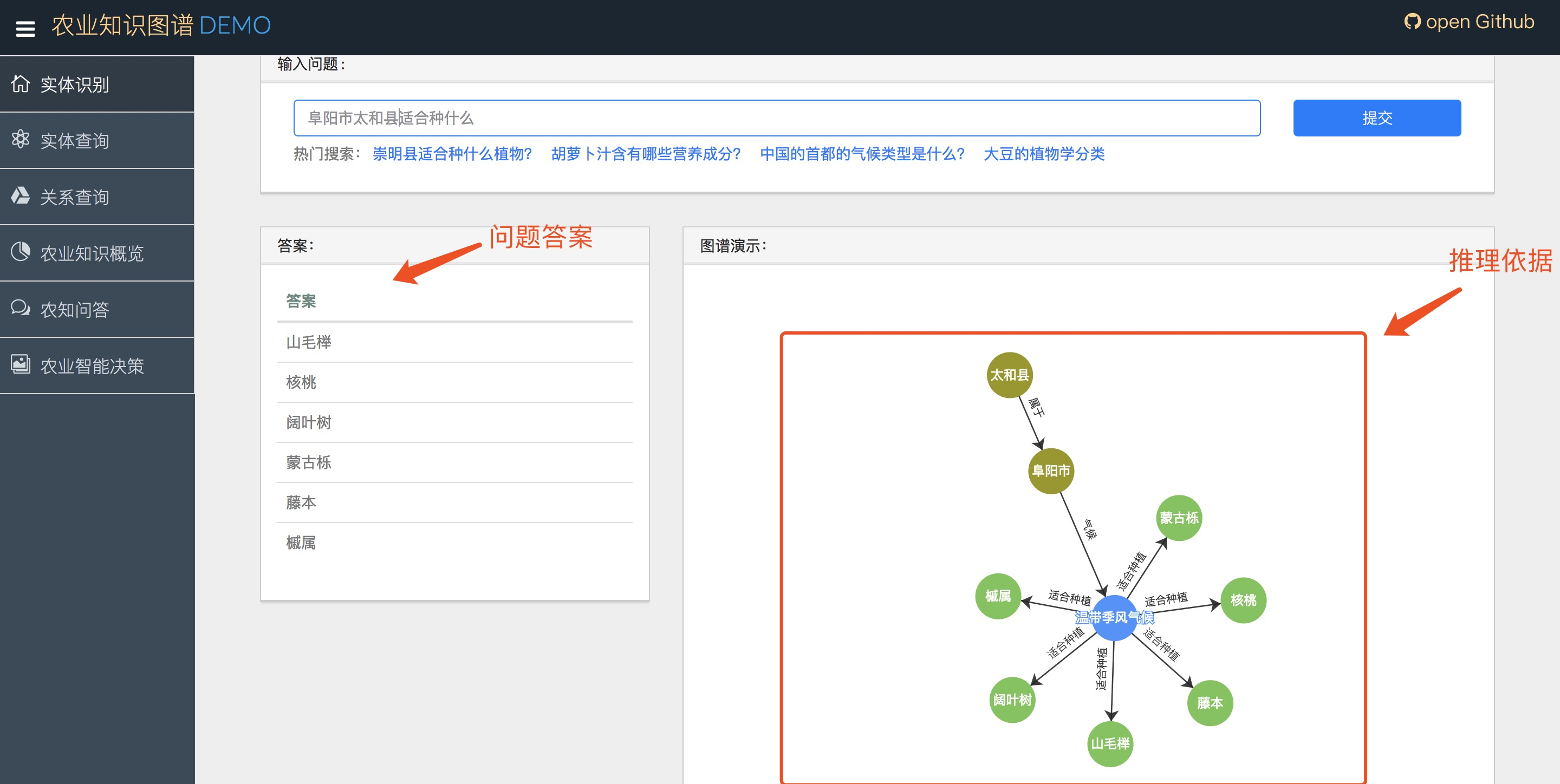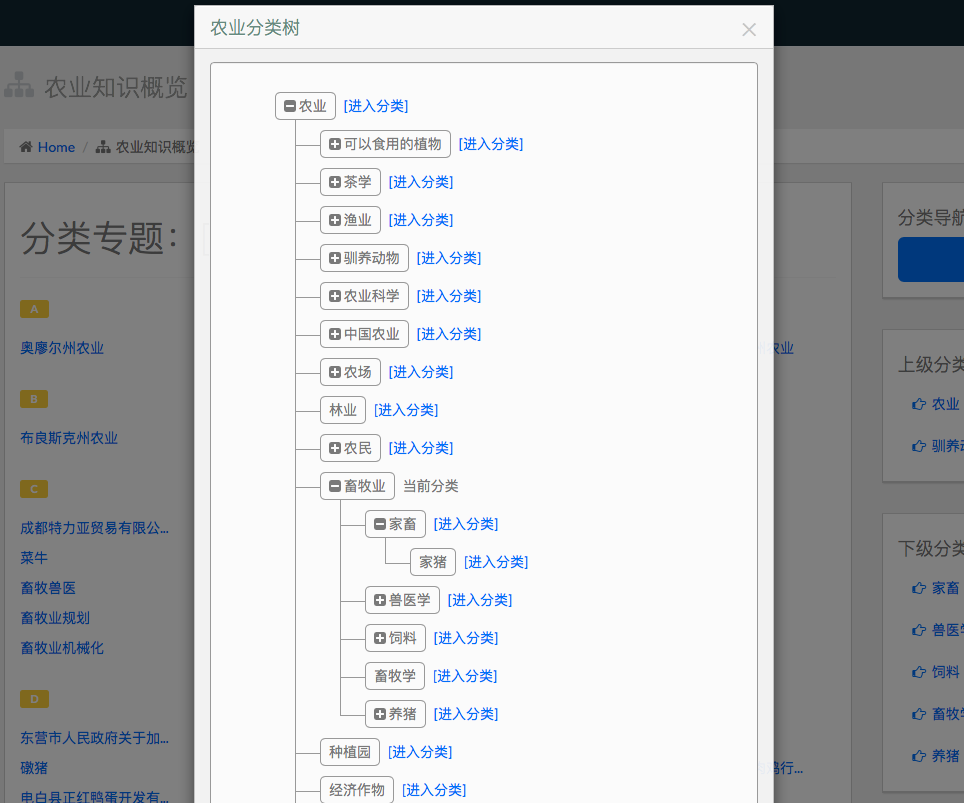十九、项目实例2：智学AI·基于深度学习的学科知识图谱
本项目为博主的本科毕业设计，网站网址为：https://www.wjn1996.cn/zxai/index。项目截图如下：
管理系统：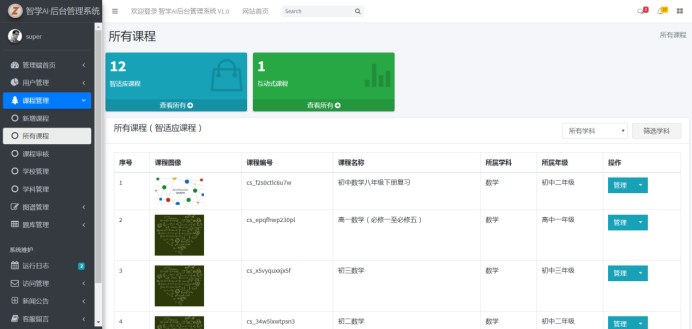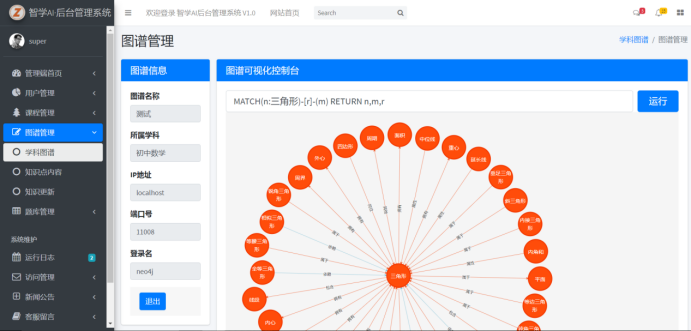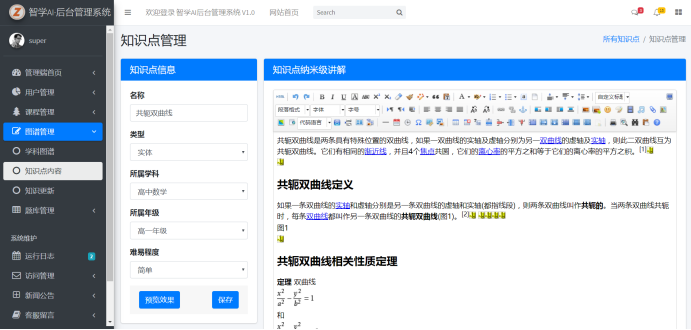学生平台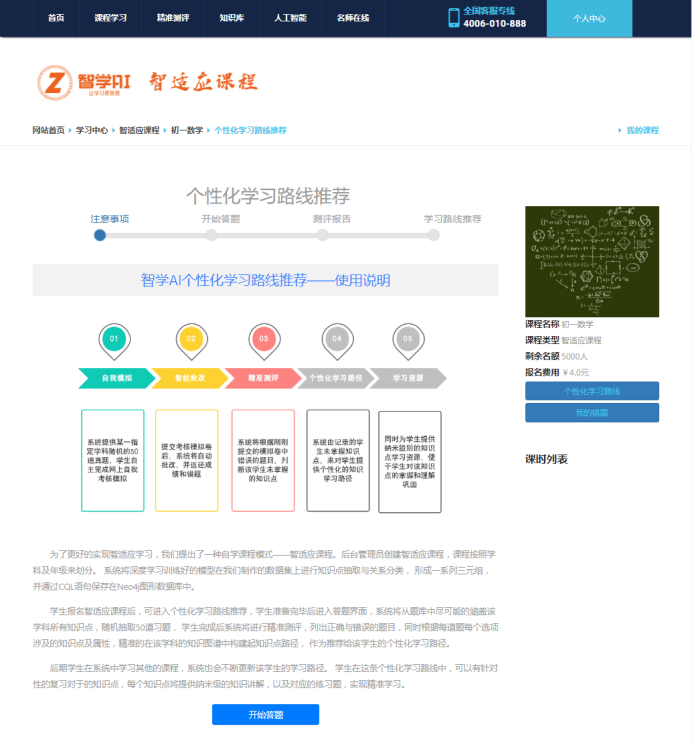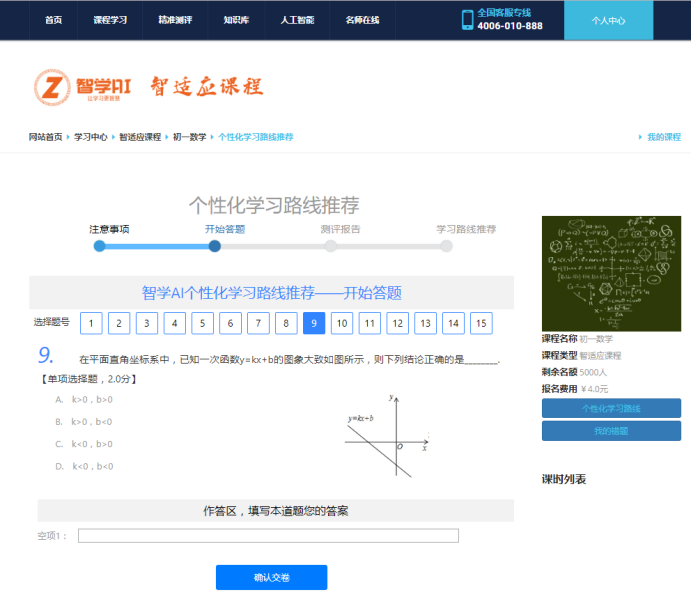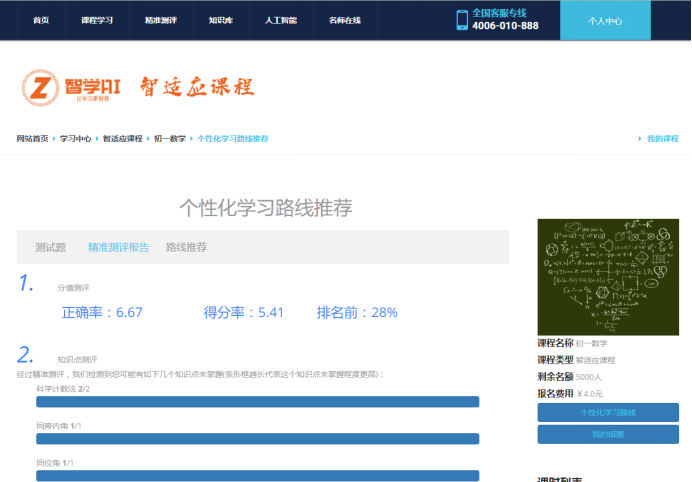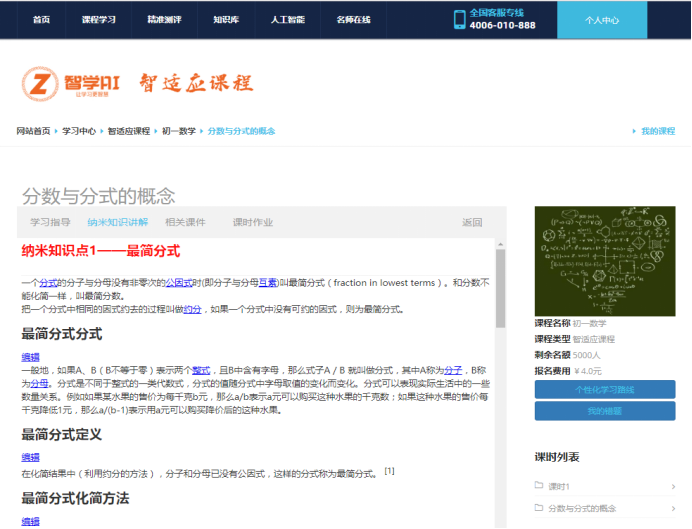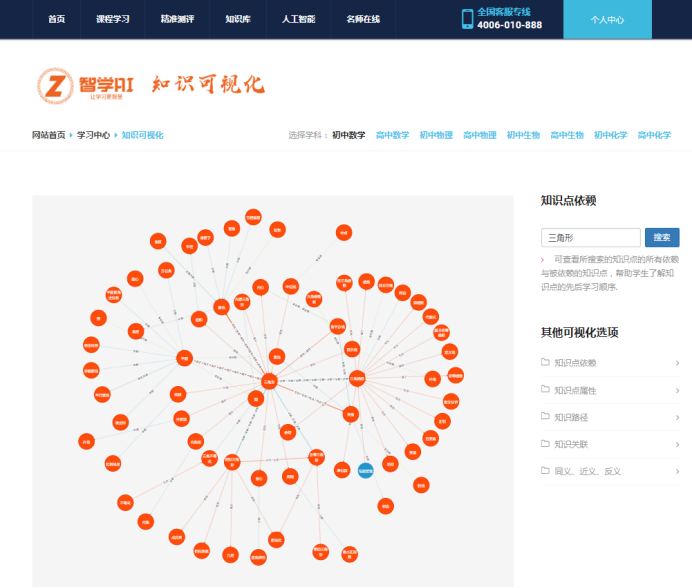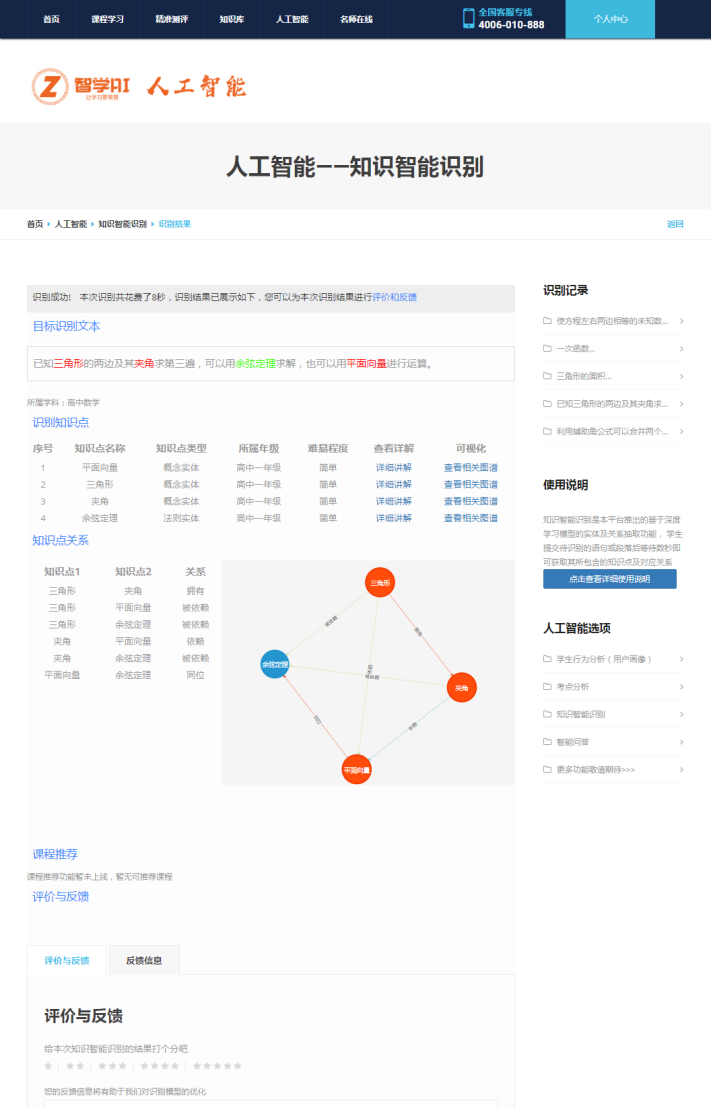二十、总结
本文章（专栏）讲述了基于深度学习的命名实体识别与关系抽取，细致的介绍了相关概念、涉及的模型以及通过公式推导和例子来对模型进行分析，最后给出源码和项目实例。
本人在研究知识图谱过程中，对知识图谱的关键步骤——知识抽取花了大量的时间，研究了深度神经网络模型、循环神经网络模型，长期记忆模型，在了解相关模型后认识到了编码解码问题，而神经网络充当着编码，因此解决解码问题就用到了概率图模型（当然LSTM也可以解码）。
命名实体识别问题可以被认为是序列标注问题，关系抽求可以被认为是监督分类问题。在2017年几篇端到端模型的文章纷纷呈现，打破了传统的pipeline模型，在实验效果上非常出色。几种端到端模型包括Bi-LSTM+LSTM+Text-CNN，Bi-LSTM+LSTM，Bi-LSTM+Independency-Tree等，这些模型目的是在命名实体抽取的过程中一并将关系挖掘出来，因此在模型的运行效率上有很大的改善。
除了端到端模型，一种基于注意力机制的模型映入眼帘，在几篇学术论文中声称其模型比端到端模型更优，在实验中也得到了体现。基于注意力机制模型还需要进行深入的研究。
关于基于深度学习的知识抽取，本文便到此结束，今后会不断根据学术论文的情况进行更新或添加。

博主同文：基于深度学习的命名实体识别与关系抽取；更多文章可点击阅读：夏栀的博客


展开全文• 远程监督关系抽取，53类关系数据，采用的都是一些主流方法，比如cnn+att,pcnn+att,ResNet+att等
• 时序关系抽取论文，令牌序列经常被用作卷积神经网络的输入。 （CNNs）在自然语言处理中的应用。 然而，它们可能不是时间表达式的理想表示，它们是 长，高度变化，语义复杂。我们描述了一种用于表示CNS的单伪令牌的...
• 以釜山行里面的人物关系为实例，为大家介绍一下人物关系抽取怎么做。里面包含代码和数据，亲测可用！
• 关系抽取 概念 从文本中识别出两个实体（或多个实体）之间存在的事实上的关系 意义 搜索引擎发现和关联知识的重要渠道 知识库构建与知识关联的基础性手段 支持问答系统、推荐系统等应用的有力工具 形式化表达 二元...
关系抽取
概念
从文本中识别出两个实体（或多个实体）之间存在的事实上的关系
意义

搜索引擎发现和关联知识的重要渠道
知识库构建与知识关联的基础性手段
支持问答系统、推荐系统等应用的有力工具

形式化表达
二元组<subject, objects> 适合特定领域关系抽取
三元组<subject, predicate, object> 适合多类型关系抽取
多元组<subject, predicate, object, time>
抽取方法
规则
模式
机器学习

事件抽取
概念
事件是信息的一种表现形式，其定义为特定的人、物，在特定时间和特定地
点相互作用所产生的客观事实.
基本要素
事件触发词：表示事件发生的核心词，多为动词或名词
事件类型：与触发词相对应，往往可以通过触发词分类加以识别
事件元素：事件的参与者，主要由实体、时间等组成.
事件元素角色：事件元素在事件中充当的角色.


展开全文• 根据参与实体的多少可以分为二元关系抽取（两个实体）和多元关系抽取（三个及以上实体）。 通过关注两个实体间的语义关系，可以得到（arg1, relation, arg2）三元组，其中arg1和arg2表示两个实体，relation表示实体...
目录

实体抽取（实体命名，NER）

关系抽取

关系抽取方法

事件抽取及方法

实体抽取（实体命名，NER）

参考：实体关系抽取一

参考：HMM、CRF、LSTM+CRF

参考：读书笔记：关系抽取和事件抽取

关系抽取

定义：自动识别实体之间具有的某种语义关系。根据参与实体的多少可以分为二元关系抽取（两个实体）和多元关系抽取（三个及以上实体）。

通过关注两个实体间的语义关系，可以得到（arg1, relation, arg2）三元组，其中arg1和arg2表示两个实体，relation表示实体间的语义关系。（比如通过Hanlp分析工具可以得到句子中各词之间的语义关系）

抽取数据源分类：

面向结构化文本的关系抽取：包括表格文档、XML文档、数据库数据等

面向非结构化文本的关系抽取：纯文本

面向半结构化文本的关系抽取：介于结构化和非结构化之间

抽取范围分类：

句子级关系抽取：从一个句子中判别两个实体间是何种语义关系
语料（篇章）级关系抽取：不限定两个目标实体所出现的上下文
抽取领域分类：

限定域关系抽取：在一个或者多个限定的领域内对实体间的语义关系进行抽取，限定关系的类别，可看成是一个文本分类任务
开放域关系抽取：不限定关系的类别
关系抽取方法

限定域关系抽取方法：

基于模板的关系抽取方法：通过人工编辑或者学习得到的模板对文本中的实体关系进行抽取和判别，受限于模板的质量和覆盖度，可扩张性不强。（自己做的法院文书属于基于模板的抽取）

基于机器学习的关系抽取方法：将关系抽取看成是一个分类问题

其中，基于机器学习的关系抽取方法又可分为 有监督 和 弱监督。

有监督的关系抽取方法：

基于特征工程的方法：需要显示地将关系实例转换成分类器可以接受的特征向量

基于核函数的方法：直接以结构树为处理对象，在计算关系之间距离的时候不再使用特征向量的内积而是用核函数

基于神经网络的方法：直接从输入的文本中自动学习有效的特征表示，端到端

弱监督的关系抽取方法：不需要人工标注大量数据。

距离监督：用开放知识图谱自动标注训练样本，不需要人工逐一标注，属弱监督关系抽取的一种。

开放域关系抽取方法：

不需要预先定义关系类别，使用实体对上下文中的一些词语来描述实体之间的关系。

事件抽取及方法

定义：从描述事件信息的文本中抽取出用户感兴趣的事件并以结构化的形式呈现出来。

步骤：首先识别出事件及其类型，其次要识别出事件所涉及的元素（一般是实体），最后需要确定每个元素在事件中所扮演的角色。

事件抽取相关概念：

事件指称：对一个客观发生的具体事件进行的自然语言形式的描述，通常是一个句子或句群
事件触发词：指一个事件指称中最能代表事件发生的词，是决定事件类别的重要特征，一般是动词或名词
事件元素：事件中的参与者，主要由实体、时间和属性值组成
元素角色：事件元素在相应的事件中扮演什么角色
事件类别：事件元素和触发词决定了事件的类别（类别又定义了若干子类别）
限定域事件抽取：在进行抽取之前，预先定义好目标事件的类型及每种类型的具体结构（包含哪些具体的事件元素），通常会给出一定数量的标注数据。

限定域事件抽取方法：

基于模式匹配的方法：对某种类型事件的识别和抽取是在一些模式的指导下进行的（步骤：模式获取、模式匹配）
有监督的事件模式匹配：模式的获取完全基于人工标注的语料
弱监督的事件模式匹配：不需要对语料进行完全标注，只需要人工对语料进行一定的预分类或者制定少量种子模式

基于机器学习的方法
有监督事件抽取方法：将事件抽取建模成一个多分类问题
基于特征工程的方法：需要显示地将事件实例转换成分类器可以接受的特征向量，研究重点在于怎样提取具有区分性的特征
基于神经网络的方法：自动从文本中获取特征进而完成事件抽取，避免使用传统自然语言处理工具带来的误差累积问题

弱监督事件抽取方法：不需要人工大量标注样本，但需要给出具有规范语义标签（事件类别、角色名称等）的标注训练数据
基于Bootstrapping的事件抽取：利用少部分人工标注的数据自动生成大规模标注数据（高置信度抽取结果会作为训练样本，然后再训练，不断迭代）
基于Distant Supervison的事件抽取：完全自动生成事件标注样本，利用结构化的事件知识库直接在非结构化文本中回标训练样本

开放域事件抽取：在进行事件识别之前，可能的事件类型以及事件的结构都是未知的，因此该任务通常没有标注数据，主要基于无监督的方法和分布假设理论。

分布假设理论：如果候选事件触发词或者候选事件元素具有相似的语境，那么这些候选事件触发词倾向于触发相同类型的事件，相应的候选事件元素倾向于扮演相同的事件元素。

开放域事件抽取方法：

基于内容特征的事件抽取方法
基于异常检测的事件抽取方法
事件关系抽取，以事件为基本语义单元，实现事件逻辑关系的深层检测和抽取，包括：

事件共指关系抽取
事件因果关系抽取
子事件关系抽取
事件时序关系抽取
总结：

组织和构建同时包含实体、实体关系、事件、事件关系的事件知识图谱得到了越来越多的关注。

展开全文• 1.关系抽取现有技术分析 1.1 基于深度学习的关系抽取任务 在基于深度学习的关系抽取任务中，其主要分为3个研究方向：（1）引入远程监督的关系抽取任务；（2）引入多示例学习的关系抽取任务；（3）联合实体识别的关系...
• ## 关系抽取综述

千次阅读 2018-06-02 16:13:54
1引言 关系抽取是信息抽取的重要子任务，其主要目的是将非结构化或半结构化描述的自然语言文本转化成结构化数据，关系抽取主要负责从文本中识别出实体，抽取实体间的语义关系。现有主流关系抽取技术分为有监督关系...关系分类 信息抽取 远程监督
• 关系抽取 (Relation Extraction, RE) 是从自然语言文本中抽取实体及其之间关系的信息技术，是信息检索、智能问答、智能对话等人工智能应用的重要基础，一直受到业界的广泛关注。关系抽取任务涉及命名实体识别、指代...
• 这是2018年CCKS会议上的关系抽取的综述性tutorial，对学习知识图谱意义重大
• 关系抽取技术研究概况进行总结。在回顾关系抽取发展历史的基础上，将关系抽取研究划分为两个阶 段: 面向特定领域的关系抽取研究和面向开放互联网文本的关系抽取研究。在分析相关文献的基础上， 总结出两 个研究...paper
• 信息抽取主要包括3项子任务：实体抽取、关系抽取和事件抽取,而关系抽取是信息抽取领域的核心任务和重要环节.实体关系抽取的主要目标是从自然语言文本中识别并判定实体对之间存在的特定关系，这为智能检索、语义分析...
• ChinesePersonRelationGraph ...中文人物关系知识图谱项目,内容包括中文人物关系图谱构建,基于知识库的数据回标,基于远程监督与bootstrapping方法的人物关系抽取,基于知识图谱的知识问答等应用. 项目地址:htt...知识图谱
• ## 关系抽取学习笔记

千次阅读 2017-07-03 00:28:57
什么是关系抽取关系抽取式从文本中抽取特定的关系，以及具有这种关系的实体。 目前将关系抽取划分为两个阶段研究：特定领域的传统关系抽取和开放领域的关系抽取关系抽取数据集： ACE04，05,SemEval Task8...
• 文章目录禁忌关系抽取数据来源抽取方法结果展示药物知识图谱叮当快药知识图谱(妇科)叮当快药知识图谱本体知识图谱说明数据说明 禁忌关系抽取 数据来源 寻医问药网站爬取的所有药物按照药物名称去重之后的药物数据集...
• ## 关系抽取总结

万次阅读 2018-05-30 14:40:59
2018.04.04更新：z增加对NYT+Freebase数据集的两个版本的说明对近几年(到2017)一些关系抽取/分类(Relation Extraction)的部分文献的一个简单总结。基本介绍基本定义关系抽取: 从一个句子中判断两个entity是否有关系...
• 1.关系抽取之远程监督算法综述 该文章介绍了PCNN、基于远程监督的关系抽取、多实例学习等。关系抽取之远程监督算法实体识别 事件抽取 知识图谱
• 2. 关系抽取方法 基于规则的方法 基于监督的方法 基于半监督的方法（最著名的两个算法是（booststrap（原始）和 booststrap（snowball） 基于无监督的方法 前两个方法在信息抽取中说过，这节主要说基于半监督的...
• ## 关系抽取介绍

千次阅读 2018-08-14 16:12:31
这一章，我们要介绍关系抽取的背景，即知道什么是知识图谱--关系抽取，以及相关的基本概述。 一、知识图谱 介绍关系抽取前，我们需要了解一下什么是知识图谱，知识图谱最早由谷歌提出，初衷是为了让机器具备推理...
• 摘要本文接着 知识图谱系列––命名实体识别方法,继续介绍关系抽取方法。知识图谱在构建的过程中，往往伴随着边试用，边拓展，边优化的过程，其中图谱拓展和优化就必须用到关系抽取方法来进一步抽取补充实体关系......